Published: 15 November 2014

# Nonlinear oscillations and chaotic dynamics of a supported FGM rectangular plate system under mixed excitations

Yasser S. Hamed1
1Department of Mathematics and Statistics, Faculty of Science, Taif University, El-Taif, El-Haweiah, P.O. Box 888, Zip Code 21974, Kingdom of Saudi Arabia
1Department of Engineering Mathematics, Faculty of Electronic Engineering, Menouf, 32952, Egypt
Views 86

#### Abstract

In this paper, we investigated the nonlinear oscillations, vibrations and chaotic dynamics of a simply supported rectangular plate made of functionally graded materials (FGMs) through a temperature field subjected to mixed excitations. The rectangular FGM plate system described by a coupled of nonlinear differential equations (two degree of freedom) including the quadratic and cubic nonlinear terms. The mathematical solutions of the governing equations of motion for the FGM plate derived using the perturbation method based on the power series expansion up to and including the second order approximation. The numerical simulations investigated using Runge-Kutta of fourth order using MATLAB and MAPLE programs. All different resonance cases reported and studied numerically. We applied both frequency response equations and phase-plane technique and also Lyapunov’s first method near the worst resonance cases to analyze the stability of the steady state solution of vibrating system. The effects of the different parameters of the rectangular plate system studied numerically. Results compared to previously published work. In the future work, we can deal with the same system subjected multi-external and tuned and parametric excitation forces. Also, the system can be studied at another worst different resonance cases, active and passive controller.

## 2. Mathematical analysis

A simply supported FGM rectangular plate subjected to a through-thickness temperature field together with tuned, parametric and external excitations is considered in this study. The plate is of length $a$, width $b$, and thickness $h$ as shown in Fig. 1. Assume that ($u\text{,}v\text{,}w$) represent the displacements of an arbitrary point of the FGM rectangular plate in the $x$, $y$, and $z$ directions respectively. Along the plate edges $x=$0, $x=a$, the plate is subjected to an in-plane excitation $p={p}_{0}-{p}_{1}\mathrm{c}\mathrm{o}\mathrm{s}{\gamma }_{2\text{\hspace{0.17em}}}t$ where ${p}_{0}$, ${p}_{1}$ are the external excitation amplitude in $y$ direction and a transverse excitation $F\left(x,y\right)\left(\mathrm{c}\mathrm{o}\mathrm{s}{\gamma }_{1}\text{\hspace{0.17em}}t+\mathrm{s}\mathrm{i}\mathrm{n}{\gamma }_{3}\text{\hspace{0.17em}}t*\mathrm{c}\mathrm{o}\mathrm{s}{\gamma }_{4}\text{\hspace{0.17em}}t\right)$, where $F\left(x,y\right)$ are the parametric and tuned excitation amplitudes in $z$ direction. Here ${\varphi }_{x}$ and ${\varphi }_{y}$ represent the mid-plane rotations of a transverse normal about the $y$ and $x$ axes, ${\gamma }_{1}$, ${\gamma }_{2}$, ${\gamma }_{3}$ and ${\gamma }_{4}$ are the excitation frequencies of the transverse and in-plane excitations, respectively. According to the Reddy’s third-order shear deformation plate theory (TSDT) , the displacement field of the plate is assumed as:

1a
$u\left(x,y,t\right)={u}_{0}\left(x,y,t\right)+z{\varphi }_{x}\left(x,y,t\right)-{c}_{1}{z}^{3}\left({\varphi }_{x}+\frac{\partial {w}_{0}}{\partial x}\right),$
1b
$v\left(x,y,t\right)={v}_{0}\left(x,y,t\right)+z{\varphi }_{y}\left(x,y,t\right)-{c}_{1}{z}^{3}\left({\varphi }_{y}+\frac{\partial {w}_{0}}{\partial y}\right),$
1c
$w\left(x,y,t\right)={w}_{0}\left(x,y,t\right),$

where (${u}_{0},{v}_{0},{w}_{0}$) represent the displacements of a point in middle plane of the FGM rectangular plate in the $x$, $y$, and $z$ directions respectively. According to the von Karman strain-displacements relationship and the Hamilton’s principle, the nonlinear governing equations of motion for the FGM rectangular plate are derived as:

2a
${N}_{xx,x}+{N}_{xy,y}={I}_{0}{\stackrel{¨}{u}}_{0}+\left({I}_{1}-{c}_{1}{I}_{3}\right){\stackrel{¨}{\varphi }}_{x}-{c}_{1}{I}_{3}\frac{\partial {\stackrel{¨}{w}}_{0}}{\partial x},$
2b
${N}_{yy,y}+{N}_{xy,y}={I}_{0}{\stackrel{¨}{v}}_{0}+\left({I}_{1}-{c}_{1}{I}_{3}\right){\stackrel{¨}{\varphi }}_{y}-{c}_{1}{I}_{3}\frac{\partial {\stackrel{¨}{w}}_{0}}{\partial y},$
2c
${N}_{yy,y}\frac{\partial {w}_{0}}{\partial y}+{N}_{yy}\frac{{\partial }^{2}{w}_{0}}{\partial {y}^{2}}+{N}_{xy,y}\frac{\partial {w}_{0}}{\partial x}+{N}_{xy,x}\frac{\partial {w}_{0}}{\partial y}+2{N}_{xy}\frac{{\partial }^{2}{w}_{0}}{\partial y\partial x}+{N}_{xx,x}\frac{\partial {w}_{0}}{\partial x}+{N}_{xx}\frac{{\partial }^{2}{w}_{0}}{\partial {x}^{2}}$
$+{c}_{1}\left({p}_{xx,xx}+2{p}_{xy,xy}+{p}_{yy,yy}\right)+\left({Q}_{x,x}-{c}_{2}{R}_{x,x}\right)+\left({Q}_{y,y}-{c}_{2}{R}_{y,y}\right)+F-\gamma {\stackrel{˙}{w}}_{0}$
$={I}_{0}{\stackrel{¨}{w}}_{0}+{c}_{1}{I}_{3}\left(\frac{\partial {\stackrel{¨}{u}}_{0}}{\partial x}+\frac{\partial {\stackrel{¨}{v}}_{0}}{\partial y}\right)+\left({c}_{1}{I}_{4}-{c}_{1}^{2}{I}_{6}\right)\left(\frac{\partial {\stackrel{¨}{\varphi }}_{x}}{\partial x}+\frac{\partial {\stackrel{¨}{\varphi }}_{y}}{\partial y}\right)-{c}_{1}^{2}{I}_{6}\left(\frac{{\partial }^{2}{\stackrel{¨}{w}}_{0}}{\partial {x}^{2}}+\frac{{\partial }^{2}{\stackrel{¨}{w}}_{0}}{\partial {y}^{2}}\right),$
2d
${M}_{xx,x}+{M}_{xy,y}-{c}_{1}\left({p}_{xx,x}+{p}_{xy,y}\right)-\left({Q}_{x}-{c}_{2}{R}_{x}\right)=\left({I}_{1}-{c}_{1}{I}_{3}\right)\text{\hspace{0.17em}}{\stackrel{¨}{u}}_{0}$
$+\left({I}_{2}-2{c}_{1}{I}_{4}+{c}_{1}^{2}{I}_{6}\right)\text{\hspace{0.17em}}{\stackrel{¨}{\varphi }}_{x}-\left({c}_{1}{I}_{4}-{c}_{1}^{2}{I}_{6}\right)\frac{\partial {\stackrel{¨}{w}}_{0}}{\partial x},$
2e
${M}_{yy,y}+{M}_{xy,y}-{c}_{1}\left({p}_{yy,y}+{p}_{xy,x}\right)-\left({Q}_{y}-{c}_{2}{R}_{y}\right)=\left({I}_{1}-{c}_{1}{I}_{3}\right)\text{\hspace{0.17em}}{\stackrel{¨}{v}}_{0}$
$+\left({I}_{2}-2{c}_{1}{I}_{4}+{c}_{1}^{2}{I}_{6}\right)\text{\hspace{0.17em}}{\stackrel{¨}{\varphi }}_{y}-\left({c}_{1}{I}_{4}-{c}_{1}^{2}{I}_{6}\right)\frac{\partial {\stackrel{¨}{w}}_{0}}{\partial y}.$

The boundary conditions for the simply supported plate under current consideration require at:

3a
$x=0,\mathrm{}\mathrm{}\mathrm{}x=a,w={\varphi }_{y}={M}_{xx}={p}_{xx}={N}_{xy}=0,$
3b
$y=0,\mathrm{}\mathrm{}\mathrm{}y=b,w={\varphi }_{x}={M}_{yy}={p}_{yy}={N}_{xy}=0,$
3c
${N}_{yy}{|}_{y=0,b}=0,\text{\hspace{0.17em}\hspace{0.17em}\hspace{0.17em}\hspace{0.17em}\hspace{0.17em}}\underset{0}{\overset{b}{\int }}{N}_{xx}\text{\hspace{0.17em}}{|}_{x=0,a}dy=-\underset{0}{\overset{b}{\int }}\left({p}_{0}-{p}_{1}\mathrm{c}\mathrm{o}\mathrm{s}{\gamma }_{2}t\right)dy.$

Fig. 1The model of a rectangular FGM plate and the coordinate system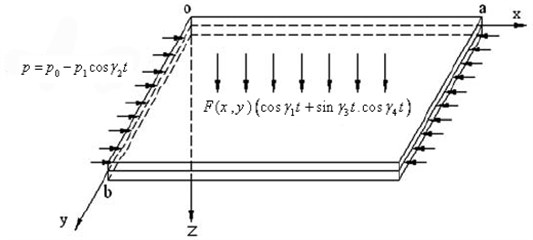Applying the Galerkin procedure yield the dimensionless governing differential equation of transverse motion of the FGM rectangular plate with two degrees of freedom as follows:

The governing differential equation of transverse motion of the FGM rectangular plate with two degrees of freedom as follows:

4a
$\stackrel{¨}{x}+{\omega }_{1}^{2}x=\epsilon \left(-{\beta }_{1}{x}^{2}-{\beta }_{2}{y}^{2}-{\beta }_{3}{x}^{3}-{\beta }_{4}x{y}^{2}-{\beta }_{5}xy\right)$
$+{\epsilon }^{2}\left({\rho }_{1}\mathrm{c}\mathrm{o}\mathrm{s}{\gamma }_{1}t-{\rho }_{11}x\mathrm{c}\mathrm{o}\mathrm{s}{\gamma }_{2}t+{\rho }_{21}\mathrm{s}\mathrm{i}\mathrm{n}{\gamma }_{3}t*\mathrm{c}\mathrm{o}\mathrm{s}{\gamma }_{4}t-{\mu }_{1}\stackrel{˙}{x}\right),$
4b
$\stackrel{¨}{y}+{\omega }_{2}^{2}y=\epsilon \left(-{\eta }_{1}{y}^{2}-{\eta }_{2}{x}^{2}-{\eta }_{3}{y}^{3}-{\eta }_{4}{x}^{2}y-{\eta }_{5}xy\right)$
$+{\epsilon }^{2}\left({\rho }_{2}\mathrm{c}\mathrm{o}\mathrm{s}{\gamma }_{1}t-{\rho }_{12}y\mathrm{c}\mathrm{o}\mathrm{s}{\gamma }_{2}t+{\rho }_{22}\mathrm{s}\mathrm{i}\mathrm{n}{\gamma }_{3}t*\mathrm{c}\mathrm{o}\mathrm{s}{\gamma }_{4}t-{\mu }_{2}\stackrel{˙}{y}\right).$

The initial conditions for the simply supported plate are:

5
$x\left(t=0\right)=0,\mathrm{}\mathrm{}\mathrm{}y\left(t=0\right)=0,\mathrm{}\mathrm{}\mathrm{}\stackrel{˙}{x}\left(t=0\right)=0,\mathrm{}\mathrm{}\mathrm{}\stackrel{˙}{y}\left(t=0\right)=0,$

where $x$ and $y$ are the amplitudes of the FGM rectangular plate for the first and second modes, ${\omega }_{1}$ and ${\omega }_{2}$ the natural frequencies of the rectangular plate modes, ${\mu }_{1}$ and ${\mu }_{2}$ are the modal damping coefficients, and ${\gamma }_{1}$, ${\gamma }_{2}$, ${\gamma }_{3}$ and ${\gamma }_{4}$ are the external and parametric and tuned excitation frequencies respectively. ${\rho }_{1}$, ${\rho }_{2}$, ${\rho }_{11}$, ${\rho }_{12}$, ${\rho }_{21}$ and ${\rho }_{22}$ are related to the magnitude of the excitation forces of the FGM rectangular plate modes, $\epsilon$ is a small perturbation parameter and $0<\epsilon <<1$. We introducing three time scales:

6
${T}_{0}=t,{T}_{1}=\epsilon t,{T}_{2}={\epsilon }^{2}t.$

We seek an asymptotic solution of Eq. (4) for $x$ and $y$ in the form:

7a
$x\left(t,\text{\hspace{0.17em}}\epsilon \right)={x}_{10}\left({T}_{0},\text{\hspace{0.17em}}{T}_{1},\text{\hspace{0.17em}}{T}_{2}\right)+\epsilon {x}_{11}\left({T}_{0},\text{\hspace{0.17em}}{T}_{1},\text{\hspace{0.17em}}{T}_{2}\right)+{\epsilon }^{2}{x}_{12}\left({T}_{0},\text{\hspace{0.17em}}{T}_{1},\text{\hspace{0.17em}}{T}_{2}\right)+O\left({\epsilon }^{3}\right),$
7b
$y\left(t,\text{\hspace{0.17em}}\epsilon \right)={y}_{10}\left({T}_{0},\text{\hspace{0.17em}}{T}_{1},\text{\hspace{0.17em}}{T}_{2}\right)+\epsilon {y}_{11}\left({T}_{0},\text{\hspace{0.17em}}{T}_{1},\text{\hspace{0.17em}}{T}_{2}\right)+{\epsilon }^{2}{y}_{12}\left({T}_{0},\text{\hspace{0.17em}}{T}_{1},\text{\hspace{0.17em}}{T}_{2}\right)+O\left({\epsilon }^{3}\right).$

Derivatives with respect to $t$ are then transformed into:

8a
$\frac{d}{dt}={D}_{0}+\epsilon \text{\hspace{0.17em}}{D}_{1}+{\epsilon }^{2}\text{\hspace{0.17em}}{D}_{2}\text{\hspace{0.17em}},$
8b
$\frac{{d}^{2}}{d{t}^{2}}={D}_{0}^{2}+2\epsilon {D}_{0}\text{\hspace{0.17em}}{D}_{1}+{\epsilon }^{2}\left(\text{\hspace{0.17em}}{D}_{1}^{2}+2{D}_{0}{D}_{2}\right).$

The derivatives $\stackrel{˙}{x}$, $\text{\hspace{0.17em}}\stackrel{˙}{y}$, $\stackrel{¨}{x}$ and $\stackrel{¨}{y}$ are in the form:

9a
$\stackrel{˙}{x}\text{\hspace{0.17em}}={D}_{0}{x}_{10}+\epsilon \left({D}_{0}{x}_{11}+{D}_{1}{x}_{10}\right)+{\epsilon }^{2}\left({D}_{0}{x}_{12}+{D}_{1}{x}_{11}+{D}_{2}{x}_{10}\right),$
9b
$\stackrel{¨}{x}={D}_{0}^{2}{x}_{10}+\epsilon \left({D}_{0}^{2}{x}_{11}+2{D}_{0}{D}_{1}{x}_{10}\right)+{\epsilon }^{2}\left({D}_{0}^{2}{x}_{12}+2{D}_{0}{D}_{1}{x}_{11}+{D}_{1}^{2}{x}_{10}+2{D}_{0}{D}_{2}{x}_{10}\right),$
9c
$\stackrel{˙}{y}\text{\hspace{0.17em}}={D}_{0}{y}_{10}+\epsilon \left({D}_{0}{y}_{11}+{D}_{1}{y}_{10}\right)+{\epsilon }^{2}\left({D}_{0}{y}_{12}+{D}_{1}{y}_{11}+{D}_{2}{y}_{10}\right),$
9d
$\stackrel{¨}{y}={D}_{0}^{2}{y}_{10}+\epsilon \left({D}_{0}^{2}{y}_{11}+2{D}_{0}{D}_{1}{y}_{10}\right)+{\epsilon }^{2}\left({D}_{0}^{2}{y}_{12}+2{D}_{0}{D}_{1}{y}_{11}+{D}_{1}^{2}{y}_{10}+2{D}_{0}{D}_{2}{y}_{10}\right),$

where ${D}_{n}=\partial /\partial {T}_{n}$, $n=$0, 1, 2. Terms of $O\left({\epsilon }^{3}\right)$ and higher orders are neglected. Substituting Eqs. (7)-(9) into Eq. (4) we get:

10a
$\left({D}_{0}^{2}{x}_{10}+\epsilon \left({D}_{0}^{2}{x}_{11}+2{D}_{0}{D}_{1}{x}_{10}\right)+{\epsilon }^{2}\left({D}_{0}^{2}{x}_{12}+2{D}_{0}{D}_{1}{x}_{11}+{D}_{1}^{2}{x}_{10}+2{D}_{0}{D}_{2}{x}_{10}\right)\right)$
$+{\omega }_{1}^{2}\left({x}_{10}+\epsilon {x}_{11}+{\epsilon }^{2}{x}_{12}\right)$
$=\epsilon \left(\begin{array}{c}-{\beta }_{1}{\left({x}_{10}+\epsilon {x}_{11}+{\epsilon }^{2}{x}_{12}\right)}^{2}-{\beta }_{2}{\left({y}_{10}+\epsilon {y}_{11}+{\epsilon }^{2}{y}_{12}\right)}^{2}\\ -{\beta }_{3}{\left({x}_{10}+\epsilon {x}_{11}+{\epsilon }^{2}{x}_{12}\right)}^{3}-{\beta }_{4}\left({x}_{10}+\epsilon {x}_{11}+{\epsilon }^{2}{x}_{12}\right){\left({y}_{10}+\epsilon {y}_{11}+{\epsilon }^{2}{y}_{12}\right)}^{2}\\ -{\beta }_{5}\left({x}_{10}+\epsilon {x}_{11}+{\epsilon }^{2}{x}_{12}\right)\left({y}_{10}+\epsilon {y}_{11}+{\epsilon }^{2}{y}_{12}\right)\end{array}\right)$
$+{\epsilon }^{2}\left(\begin{array}{c}{\rho }_{1}cos{\gamma }_{1}t-{\rho }_{11}\left({x}_{10}+\epsilon {x}_{11}+{\epsilon }^{2}{x}_{12}\right)cos{\gamma }_{2}t+{\rho }_{21}sin{\gamma }_{3}tcos{\gamma }_{4}t\\ -{\mu }_{1}\left({D}_{0}{x}_{10}+\epsilon \left({D}_{0}{x}_{11}+{D}_{1}{x}_{10}\right)+{\epsilon }^{2}\left({D}_{0}{x}_{12}+{D}_{1}{x}_{11}+{D}_{2}{x}_{10}\right)\right)\end{array}\right),$
10b
$\left({D}_{0}^{2}{y}_{10}+\epsilon \left({D}_{0}^{2}{y}_{11}+2{D}_{0}{D}_{1}{y}_{10}\right)+{\epsilon }^{2}\left({D}_{0}^{2}{y}_{12}+2{D}_{0}{D}_{1}{y}_{11}+{D}_{1}^{2}{y}_{10}+2{D}_{0}{D}_{2}{y}_{10}\right)\right)$
$+{\omega }_{2}^{2}\left({y}_{10}+\epsilon {y}_{11}+{\epsilon }^{2}{y}_{12}\right)$
$=\epsilon \left(\begin{array}{c}-{\eta }_{1}{\left({y}_{10}+\epsilon {y}_{11}+{\epsilon }^{2}{y}_{12}\right)}^{2}-{\eta }_{2}{\left({x}_{10}+\epsilon {x}_{11}+{\epsilon }^{2}{x}_{12}\right)}^{2}\\ -{\eta }_{3}{\left({y}_{10}+\epsilon {y}_{11}+{\epsilon }^{2}{y}_{12}\right)}^{3}-{\eta }_{4}{\left({x}_{10}+\epsilon {x}_{11}+{\epsilon }^{2}{x}_{12}\right)}^{2}\left({y}_{10}+\epsilon {y}_{11}+{\epsilon }^{2}{y}_{12}\right)\\ -{\eta }_{5}\left({x}_{10}+\epsilon {x}_{11}+{\epsilon }^{2}{x}_{12}\right)\left({y}_{10}+\epsilon {y}_{11}+{\epsilon }^{2}{y}_{12}\right)\end{array}\right)$
$+{\epsilon }^{2}\left(\begin{array}{c}{\rho }_{2}cos{\gamma }_{1}t-{\rho }_{12}\left({y}_{10}+\epsilon {y}_{11}+{\epsilon }^{2}{y}_{12}\right)cos{\gamma }_{2}t+{\rho }_{22}sin{\gamma }_{3}tcos{\gamma }_{4}t\\ -{\mu }_{2}\left({D}_{0}{y}_{10}+\epsilon \left({D}_{0}{y}_{11}+{D}_{1}{y}_{10}\right)+{\epsilon }^{2}\left({D}_{0}{y}_{12}+{D}_{1}{y}_{11}+{D}_{2}{y}_{10}\right)\right)\end{array}\right).$

We expand the two sides of Eqs. (10) and equating the coefficients of similar powers of $\epsilon$, one obtains the following set of ordinary differential equations:

Order ${\epsilon }^{0}$:

11a
$\left({D}_{0}^{2}+{\omega }_{1}^{2}\right)\text{\hspace{0.17em}}{x}_{10}=0,$
11b
$\left({D}_{0}^{2}+{\omega }_{2}^{2}\right)\text{\hspace{0.17em}}{y}_{10}=0.$

Order ${\epsilon }^{1}$:

12a
$\left({D}_{0}^{2}+{\omega }_{1}^{2}\right)\text{\hspace{0.17em}}{x}_{11}=-2{D}_{0}{D}_{1}\text{\hspace{0.17em}}{x}_{10}-{\beta }_{1}\text{\hspace{0.17em}}{x}_{10}^{2}-{\beta }_{2}\text{\hspace{0.17em}}{y}_{10}^{2}-{\beta }_{3}\text{\hspace{0.17em}}{x}_{10}^{3}-{\beta }_{4}\text{\hspace{0.17em}}{x}_{10}\text{\hspace{0.17em}}{y}_{10}^{2}-{\beta }_{5}\text{\hspace{0.17em}}{x}_{10}\text{\hspace{0.17em}}{y}_{10},$
12b
$\left({D}_{0}^{2}+{\omega }_{2}^{2}\right)\text{\hspace{0.17em}}{y}_{11}=-2{D}_{0}{D}_{1}\text{\hspace{0.17em}}{y}_{10}-{\eta }_{1}\text{\hspace{0.17em}}{y}_{10}^{2}-{\eta }_{2}\text{\hspace{0.17em}}{x}_{10}^{2}-{\eta }_{3}\text{\hspace{0.17em}}{y}_{10}^{3}-{\eta }_{4}\text{\hspace{0.17em}}{y}_{10}{x}_{10}^{2}-{\eta }_{5}\text{\hspace{0.17em}}{y}_{10}\text{\hspace{0.17em}}{x}_{10}.$

Order ${\epsilon }^{2}$:

13a
$\left({D}_{0}^{2}+{\omega }_{1}^{2}\right)\text{\hspace{0.17em}}{x}_{12}=-2{D}_{0}\text{\hspace{0.17em}}{D}_{2}\text{\hspace{0.17em}}{x}_{10}-{D}_{1}^{2}{x}_{10}-2{D}_{0}{D}_{1}\text{\hspace{0.17em}}{x}_{11}-2{\beta }_{1}\text{\hspace{0.17em}}{x}_{10}\text{\hspace{0.17em}}{x}_{11}-2{\beta }_{2}\text{\hspace{0.17em}}{y}_{10}\text{\hspace{0.17em}}{y}_{11}$
$-3{\beta }_{3}\text{\hspace{0.17em}}{x}_{10}^{2}\text{\hspace{0.17em}}{x}_{11}-{\mu }_{1}{D}_{0}{x}_{10}-{\beta }_{4}\left({x}_{11}\text{\hspace{0.17em}}{y}_{10}^{2}+2{x}_{10}\text{\hspace{0.17em}}{y}_{10}\text{\hspace{0.17em}}{y}_{11}\right)-{\beta }_{5}\left({x}_{10\text{\hspace{0.17em}}}{y}_{11}+{x}_{11}\text{\hspace{0.17em}}{y}_{10}\right)$
$-{\rho }_{11}{x}_{10\text{\hspace{0.17em}}}\mathrm{c}\mathrm{o}\mathrm{s}{\gamma }_{2\text{\hspace{0.17em}}}t\text{\hspace{0.17em}}+{\rho }_{1}\mathrm{c}\mathrm{o}\mathrm{s}{\gamma }_{1}\text{\hspace{0.17em}}t+{\rho }_{21}\mathrm{s}\mathrm{i}\mathrm{n}{\gamma }_{3}\text{\hspace{0.17em}}t\mathrm{c}\mathrm{o}\mathrm{s}{\gamma }_{4}\text{\hspace{0.17em}}t,$
13b
$\left({D}_{0}^{2}+{\omega }_{2}^{2}\right)\text{\hspace{0.17em}}{y}_{12}=-2{D}_{0}\text{\hspace{0.17em}}{D}_{2}\text{\hspace{0.17em}}{y}_{10}-{D}_{1}^{2}{y}_{10}-2{D}_{0}{D}_{1}\text{\hspace{0.17em}}{y}_{11}-2{\eta }_{1}\text{\hspace{0.17em}}{y}_{10}\text{\hspace{0.17em}}{y}_{11}-2{\eta }_{2}\text{\hspace{0.17em}}{x}_{10}\text{\hspace{0.17em}}{x}_{11}$
$-3{\eta }_{3}\text{\hspace{0.17em}}{y}_{10}^{2}\text{\hspace{0.17em}}{y}_{11}-{\mu }_{2}{D}_{0}{y}_{10}-{\eta }_{4}\left({y}_{11}\text{\hspace{0.17em}}{x}_{10}^{2}+2{y}_{10}\text{\hspace{0.17em}}{x}_{10}\text{\hspace{0.17em}}{x}_{11}\right)-{\eta }_{5}\left({y}_{10}{x}_{11}+{y}_{11}\text{\hspace{0.17em}}{x}_{10}\right)$
$-{\rho }_{12}{y}_{10}\mathrm{c}\mathrm{o}\mathrm{s}{\gamma }_{2\text{\hspace{0.17em}}}t\text{\hspace{0.17em}}+{\rho }_{2}\mathrm{c}\mathrm{o}\mathrm{s}{\gamma }_{1}\text{\hspace{0.17em}}t+{\rho }_{22}\mathrm{s}\mathrm{i}\mathrm{n}{\gamma }_{3}\text{\hspace{0.17em}}t\mathrm{c}\mathrm{o}\mathrm{s}{\gamma }_{4}\text{\hspace{0.17em}}t.$

The general solutions of Eq. (11), can be written in the form:

14a
${x}_{10}={A}_{1}\mathrm{e}\mathrm{x}\mathrm{p}\left(i{\omega }_{1}{T}_{0}\right)+{\stackrel{-}{A}}_{1}\mathrm{e}\mathrm{x}\mathrm{p}\left(-i{\omega }_{1}{T}_{0}\right),$
14b
${y}_{10}={A}_{2}\text{\hspace{0.17em}}\mathrm{e}\mathrm{x}\mathrm{p}\left(i{\omega }_{2}{T}_{0}\right)+{\stackrel{-}{A}}_{2}\text{\hspace{0.17em}}\mathrm{e}\mathrm{x}\mathrm{p}\left(-i{\omega }_{2}{T}_{0}\right),$

where ${A}_{1}$ and ${A}_{2}$ are a complex function in ${T}_{1}$, ${T}_{2}$. Substituting Eq. (14) into Eq. (12) we get:

15a
$\left({D}_{0}^{2}+{\omega }_{1}^{2}\right)\text{\hspace{0.17em}}{x}_{11}=\left(-2{D}_{0}{D}_{1}\text{\hspace{0.17em}}{A}_{1}-2{\beta }_{4}\text{\hspace{0.17em}}{A}_{1}{A}_{2}{\stackrel{-}{A}}_{2}-3{\beta }_{3}{A}_{1}^{2}{\stackrel{-}{A}}_{1}\right)\mathrm{exp}\left(i{\omega }_{1}{T}_{0}\right)$
$+\left(-2{D}_{0}{D}_{1}\text{\hspace{0.17em}}{\stackrel{-}{A}}_{1}-2{\beta }_{4}\text{\hspace{0.17em}}{\stackrel{-}{A}}_{1}{\stackrel{-}{A}}_{2}{A}_{2}-3{\beta }_{3}{\stackrel{-}{A}}_{1}^{2}{A}_{1}\right)\mathrm{exp}\left(-i{\omega }_{1}{T}_{0}\right)-{\beta }_{1}{A}_{1}^{2}\mathrm{exp}\left(2i{\omega }_{1}{T}_{0}\right)$
$-{\beta }_{1}{\stackrel{-}{A}}_{1}^{2}\mathrm{exp}\left(-2i{\omega }_{1}{T}_{0}\right)-{\beta }_{2}{A}_{2}^{2}\mathrm{exp}\left(2i{\omega }_{2}{T}_{0}\right)-{\beta }_{2}{\stackrel{-}{A}}_{2}^{2}\mathrm{exp}\left(-2i{\omega }_{2}{T}_{0}\right)$
$-{\beta }_{3}{A}_{1}^{3}\mathrm{e}\mathrm{x}\mathrm{p}\left(3i{\omega }_{1}{T}_{0}\right)-{\beta }_{3}{\stackrel{-}{A}}_{1}^{3}\mathrm{e}\mathrm{x}\mathrm{p}\left(-3i{\omega }_{1}{T}_{0}\right)-{\beta }_{5}{A}_{1}{A}_{2}\mathrm{e}\mathrm{x}\mathrm{p}\left(i\left({\omega }_{1}+{\omega }_{2}\right){T}_{0}\right)\text{\hspace{0.17em}}$
$-{\beta }_{5}{\stackrel{-}{A}}_{1}{\stackrel{-}{A}}_{2}\mathrm{exp}\left(-i\left({\omega }_{1}+{\omega }_{2}\right){T}_{0}\right)-{\beta }_{5}{A}_{1}{\stackrel{-}{A}}_{2}\mathrm{exp}\left(i\left({\omega }_{1}-{\omega }_{2}\right){T}_{0}\right)$
$-{\beta }_{5}{\stackrel{-}{A}}_{1}{A}_{2}\mathrm{exp}\left(-i\left({\omega }_{1}-{\omega }_{2}\right){T}_{0}\right)-{\beta }_{4}{A}_{1}{A}_{2}^{2}\mathrm{exp}\left(i\left({\omega }_{1}+2{\omega }_{2}\right){T}_{0}\right)\text{\hspace{0.17em}}$
$-{\beta }_{4}{\stackrel{-}{A}}_{1}{\stackrel{-}{A}}_{2}^{2}\mathrm{exp}\left(-i\left({\omega }_{1}+2{\omega }_{2}\right){T}_{0}\right)-{\beta }_{4}{A}_{1}{\stackrel{-}{A}}_{2}^{2}\mathrm{exp}\left(i\left({\omega }_{1}-2{\omega }_{2}\right){T}_{0}\right)$
$-{\beta }_{4}{\stackrel{-}{A}}_{1}{A}_{2}^{2}\mathrm{e}\mathrm{x}\mathrm{p}\left(-i\left({\omega }_{1}-2{\omega }_{2}\right){T}_{0}\right)-2{\beta }_{1}{A}_{1}{\stackrel{-}{A}}_{1}-2{\beta }_{2}{A}_{2}{\stackrel{-}{A}}_{2},$
15b
$\left({D}_{0}^{2}+{\omega }_{2}^{2}\right)\text{\hspace{0.17em}}{y}_{11}=\left(-2{D}_{0}{D}_{1}\text{\hspace{0.17em}}{A}_{2}-2{\eta }_{4}\text{\hspace{0.17em}}{A}_{1}{\stackrel{-}{A}}_{1}{A}_{2}-3{\eta }_{3}{A}_{2}^{2}{\stackrel{-}{A}}_{2}\right)\mathrm{e}\mathrm{x}\mathrm{p}\left(i{\omega }_{2}{T}_{0}\right)$
$+\left(-2{D}_{0}{D}_{1}\text{\hspace{0.17em}}{\stackrel{-}{A}}_{2}-2{\eta }_{4}\text{\hspace{0.17em}}{\stackrel{-}{A}}_{1}{A}_{1}{\stackrel{-}{A}}_{2}-3{\eta }_{3}{A}_{2}{\stackrel{-}{A}}_{2}^{2}\right)\mathrm{e}\mathrm{x}\mathrm{p}\left(-i{\omega }_{2}{T}_{0}\right)-{\eta }_{2}{A}_{1}^{2}\mathrm{e}\mathrm{x}\mathrm{p}\left(2i{\omega }_{1}{T}_{0}\right)$
$-{\eta }_{1}{\stackrel{-}{A}}_{1}^{2}\mathrm{e}\mathrm{x}\mathrm{p}\left(-2i{\omega }_{1}{T}_{0}\right)-{\eta }_{1}{A}_{2}^{2}\mathrm{e}\mathrm{x}\mathrm{p}\left(2i{\omega }_{2}{T}_{0}\right)-{\eta }_{1}{\stackrel{-}{A}}_{2}^{2}\mathrm{e}\mathrm{x}\mathrm{p}\left(-2i{\omega }_{2}{T}_{0}\right)$
$-{\eta }_{3}{A}_{2}^{3}\mathrm{e}\mathrm{x}\mathrm{p}\left(3i{\omega }_{2}{T}_{0}\right)-{\eta }_{3}{\stackrel{-}{A}}_{2}^{3}\mathrm{e}\mathrm{x}\mathrm{p}\left(-3i{\omega }_{2}{T}_{0}\right)-{\eta }_{5}{A}_{1}{A}_{2}\mathrm{e}\mathrm{x}\mathrm{p}\left(i\left({\omega }_{2}+{\omega }_{1}\right){T}_{0}\right)\text{\hspace{0.17em}}$
$-{\eta }_{5}{\stackrel{-}{A}}_{1}{\stackrel{-}{A}}_{2}\mathrm{e}\mathrm{x}\mathrm{p}\left(-i\left({\omega }_{2}+{\omega }_{1}\right){T}_{0}\right)-{\eta }_{5}{A}_{2}{\stackrel{-}{A}}_{1}\mathrm{e}\mathrm{x}\mathrm{p}\left(i\left({\omega }_{2}-{\omega }_{1}\right){T}_{0}\right)\text{\hspace{0.17em}}$
$-{\eta }_{5}{\stackrel{-}{A}}_{2}{A}_{1}\mathrm{e}\mathrm{x}\mathrm{p}\left(-i\left({\omega }_{2}-{\omega }_{1}\right){T}_{0}\right)-{\eta }_{4}{A}_{2}{A}_{1}^{2}\mathrm{e}\mathrm{x}\mathrm{p}\left(i\left({\omega }_{2}+2{\omega }_{1}\right){T}_{0}\right)\text{\hspace{0.17em}}$
$-{\eta }_{4}{\stackrel{-}{A}}_{2}{\stackrel{-}{A}}_{1}^{2}\mathrm{e}\mathrm{x}\mathrm{p}\left(-i\left({\omega }_{2}+2{\omega }_{1}\right){T}_{0}\right)-{\eta }_{4}{A}_{2}{\stackrel{-}{A}}_{1}^{2}\mathrm{e}\mathrm{x}\mathrm{p}\left(i\left({\omega }_{2}-2{\omega }_{1}\right){T}_{0}\right)$
$-{\eta }_{4}{\stackrel{-}{A}}_{2}{A}_{1}^{2}\mathrm{e}\mathrm{x}\mathrm{p}\left(-i\left({\omega }_{2}-2{\omega }_{1}\right){T}_{0}\right)-2{\eta }_{1}{A}_{2}{\stackrel{-}{A}}_{2}-2{\eta }_{2}{A}_{1}{\stackrel{-}{A}}_{1}.$

Eliminating the secular terms ${e}^{\left(±i{\omega }_{1}{T}_{0}\right)}$ and ${e}^{\left(±i{\omega }_{2}{T}_{0}\right)}$ from Eqs. (15), then the first-order approximations are given by:

16a
${x}_{11}={K}_{1}\mathrm{e}\mathrm{x}\mathrm{p}\left(2i{\omega }_{1}{T}_{0}\right)+{K}_{2}\mathrm{e}\mathrm{x}\mathrm{p}\left(2i{\omega }_{2}{T}_{0}\right)+{K}_{3}\mathrm{e}\mathrm{x}\mathrm{p}\left(3i{\omega }_{1}{T}_{0}\right)+{K}_{4}\mathrm{e}\mathrm{x}\mathrm{p}\left[\left(i\left({\omega }_{1}+{\omega }_{2}\right){T}_{0}\right)\right]$
$+{K}_{5}\mathrm{e}\mathrm{x}\mathrm{p}\left[\left(i\left({\omega }_{1}-{\omega }_{2}\right){T}_{0}\right)\right]+{K}_{6}\mathrm{e}\mathrm{x}\mathrm{p}\left[\left(i\left({\omega }_{1}+2{\omega }_{2}\right){T}_{0}\right)\right]+{K}_{7}\mathrm{e}\mathrm{x}\mathrm{p}\left[\left(i\left({\omega }_{1}-2{\omega }_{2}\right){T}_{0}\right)\right]$
$+{K}_{8}+cc,$
16b
${y}_{11}={G}_{1}\mathrm{e}\mathrm{x}\mathrm{p}\left(2i{\omega }_{1}{T}_{0}\right)+{G}_{2}\mathrm{e}\mathrm{x}\mathrm{p}\left(2i{\omega }_{2}{T}_{0}\right)+{G}_{3}\mathrm{e}\mathrm{x}\mathrm{p}\left(3i{\omega }_{2}{T}_{0}\right)+{G}_{4}\mathrm{e}\mathrm{x}\mathrm{p}\left[\left(i\left({\omega }_{2}+{\omega }_{1}\right){T}_{0}\right)\right]$
$+{G}_{5}\mathrm{e}\mathrm{x}\mathrm{p}\left[\left(i\left({\omega }_{2}-{\omega }_{1}\right){T}_{0}\right)\right]+{G}_{6}\mathrm{e}\mathrm{x}\mathrm{p}\left[\left(i\left({\omega }_{2}+2{\omega }_{1}\right){T}_{0}\right)\right]+{G}_{7}\mathrm{e}\mathrm{x}\mathrm{p}\left[\left(i\left({\omega }_{2}-2{\omega }_{1}\right){T}_{0}\right)\right]$
$+{G}_{8}\text{\hspace{0.17em}}+cc,$

where ${K}_{i}$, ($i=$1,..., 8) and ${G}_{i}$, ($i=$1,..., 8) are a complex function in ${T}_{1}$, ${T}_{2}$ which are given in the appendix and $cc$ stands for the complex conjugate of the preceding terms.

With the same steps used to get the first-order approximation, substituting Eqs. (14), (16) into Eq. (13) and eliminating the secular terms, then the second-order approximations are given by:

17a
$\text{\hspace{0.17em}}{x}_{12}\left(\text{\hspace{0.17em}}{T}_{0\text{\hspace{0.17em}}},\text{\hspace{0.17em}}{T}_{1\text{\hspace{0.17em}}},\text{\hspace{0.17em}}{T}_{2}\right)=\text{\hspace{0.17em}\hspace{0.17em}}{E}_{1}\mathrm{e}\mathrm{x}\mathrm{p}\left({\omega }_{2}{T}_{0}\right)+{E}_{2}\mathrm{e}\mathrm{x}\mathrm{p}\left(2i{\omega }_{1\text{\hspace{0.17em}}}{T}_{0}\right)+{E}_{3}\mathrm{e}\mathrm{x}\mathrm{p}\left(2i{\omega }_{2}{T}_{0}\right)$
$+{E}_{4}\mathrm{e}\mathrm{x}\mathrm{p}\text{\hspace{0.17em}}\left(3i{\omega }_{1\text{\hspace{0.17em}}}{T}_{0}\right)+{E}_{5}\mathrm{e}\mathrm{x}\mathrm{p}\left(3i{\omega }_{2}{T}_{0}\right)+{E}_{6}\mathrm{e}\mathrm{x}\mathrm{p}\left(4i{\omega }_{1\text{\hspace{0.17em}}}{T}_{0}\right)+{E}_{7}\mathrm{e}\mathrm{x}\mathrm{p}\left(4i{\omega }_{2}{T}_{0}\right)$
$+{E}_{8}\mathrm{e}\mathrm{x}\mathrm{p}\text{\hspace{0.17em}}\left(5i{\omega }_{1\text{\hspace{0.17em}}}{T}_{0}\right)+{E}_{9}\mathrm{e}\mathrm{x}\mathrm{p}\left(i\left({\omega }_{1}+{\omega }_{2}\right)\text{\hspace{0.17em}}{T}_{0}\right)+{E}_{10}\mathrm{e}\mathrm{x}\mathrm{p}\text{\hspace{0.17em}}\left(i\left({\omega }_{1}-{\omega }_{2}\right)\text{\hspace{0.17em}}{T}_{0}\right)$
$+{E}_{11}\mathrm{e}\mathrm{x}\mathrm{p}\left(i\left({\omega }_{1}+2{\omega }_{2}\right)\text{\hspace{0.17em}}{T}_{0}\right)+{E}_{12}\mathrm{e}\mathrm{x}\mathrm{p}\left(i\left({\omega }_{1}-2{\omega }_{2}\right)\text{\hspace{0.17em}}{T}_{0}\right)+{E}_{13}\mathrm{e}\mathrm{x}\mathrm{p}\left(i\left({\omega }_{1}+3{\omega }_{2}\right)\text{\hspace{0.17em}}{T}_{0}\right)$
$+{E}_{14}\mathrm{e}\mathrm{x}\mathrm{p}\left(i\left({\omega }_{1}-3{\omega }_{2}\right)\text{\hspace{0.17em}}{T}_{0}\right)+{E}_{15}\mathrm{e}\mathrm{x}\mathrm{p}\left(i\left({\omega }_{1}+4{\omega }_{2}\right)\text{\hspace{0.17em}}{T}_{0}\right)+{E}_{16}\mathrm{e}\mathrm{x}\mathrm{p}\left(i\left({\omega }_{1}-4{\omega }_{2}\right)\text{\hspace{0.17em}}{T}_{0}\right)$
$+{E}_{17}\mathrm{e}\mathrm{x}\mathrm{p}\left(i\left(2{\omega }_{1}+{\omega }_{2}\right)\text{\hspace{0.17em}}{T}_{0}\right)+{E}_{18}\mathrm{e}\mathrm{x}\mathrm{p}\left(i\left(2{\omega }_{1}-{\omega }_{2}\right)\text{\hspace{0.17em}}{T}_{0}\right)+{E}_{19}\mathrm{e}\mathrm{x}\mathrm{p}\left(i\left(2{\omega }_{1}+2{\omega }_{2}\right)\text{\hspace{0.17em}}{T}_{0}\right)$
$+{E}_{20}\mathrm{e}\mathrm{x}\mathrm{p}\left(i\left(2{\omega }_{1}-2{\omega }_{2}\right)\text{\hspace{0.17em}}{T}_{0}\right)+{E}_{21}\mathrm{e}\mathrm{x}\mathrm{p}\left(i\left(3{\omega }_{1}+{\omega }_{2}\right)\text{\hspace{0.17em}}{T}_{0}\right)+{E}_{22}\mathrm{e}\mathrm{x}\mathrm{p}\left(i\left(3{\omega }_{1}-{\omega }_{2}\right)\text{\hspace{0.17em}}{T}_{0}\right)$
$+{E}_{23}\mathrm{e}\mathrm{x}\mathrm{p}\left(i\left(3{\omega }_{1}+2{\omega }_{2}\right)\text{\hspace{0.17em}}{T}_{0}\right)+{E}_{24}\mathrm{e}\mathrm{x}\mathrm{p}\left(i\left(3{\omega }_{1}-2{\omega }_{2}\right)\text{\hspace{0.17em}}{T}_{0}\right)+{E}_{25}\mathrm{e}\mathrm{x}\mathrm{p}\left(i\left({\gamma }_{2}+{\omega }_{1}\right)\text{\hspace{0.17em}}{T}_{0}\right)$
$+{E}_{26}\mathrm{e}\mathrm{x}\mathrm{p}\left(i\left({\gamma }_{2}-{\omega }_{1}\right)\text{\hspace{0.17em}}{T}_{0}\right)+{E}_{27}\mathrm{e}\mathrm{x}\mathrm{p}\left(i{\gamma }_{1}{T}_{0}\right)+{E}_{28}\mathrm{e}\mathrm{x}\mathrm{p}\left(i\left({\gamma }_{3}+{\gamma }_{4}\right)\text{\hspace{0.17em}}{T}_{0}\right)$
$+{E}_{29}\mathrm{e}\mathrm{x}\mathrm{p}\left(i\left({\gamma }_{3}-{\gamma }_{4}\right)\text{\hspace{0.17em}}{T}_{0}\right)+{E}_{30}+cc,$
17b
$\text{\hspace{0.17em}}{y}_{12}\left(\text{\hspace{0.17em}}{T}_{0\text{\hspace{0.17em}}},\text{\hspace{0.17em}}{T}_{1\text{\hspace{0.17em}}},\text{\hspace{0.17em}}{T}_{2}\right)=\text{\hspace{0.17em}\hspace{0.17em}}{H}_{1}\mathrm{e}\mathrm{x}\mathrm{p}\left({\omega }_{1}{T}_{0}\right)+{H}_{2}\mathrm{e}\mathrm{x}\mathrm{p}\left(2i{\omega }_{1\text{\hspace{0.17em}}}{T}_{0}\right)+{H}_{3}\mathrm{e}\mathrm{x}\mathrm{p}\left(2i{\omega }_{2}{T}_{0}\right)$
$+{H}_{4}\mathrm{e}\mathrm{x}\mathrm{p}\left(3i{\omega }_{1\text{\hspace{0.17em}}}{T}_{0}\right)+{H}_{5}\mathrm{e}\mathrm{x}\mathrm{p}\left(3i{\omega }_{2}{T}_{0}\right)+{H}_{6}\mathrm{e}\mathrm{x}\mathrm{p}\text{\hspace{0.17em}}\left(4i{\omega }_{1\text{\hspace{0.17em}}}{T}_{0}\right)+{H}_{7}\mathrm{e}\mathrm{x}\mathrm{p}\left(4i{\omega }_{2}{T}_{0}\right)$
$+{H}_{8}\mathrm{e}\mathrm{x}\mathrm{p}\left(5i{\omega }_{2}{T}_{0}\right)+{H}_{9}\mathrm{e}\mathrm{x}\mathrm{p}\left(i\left({\omega }_{1}+{\omega }_{2}\right)\text{\hspace{0.17em}}{T}_{0}\right)+{H}_{10}\mathrm{e}\mathrm{x}\mathrm{p}\text{\hspace{0.17em}}\left(i\left({\omega }_{1}-{\omega }_{2}\right)\text{\hspace{0.17em}}{T}_{0}\right)$
$+{H}_{11}\mathrm{e}\mathrm{x}\mathrm{p}\left(i\left({\omega }_{1}+2{\omega }_{2}\right)\text{\hspace{0.17em}}{T}_{0}\right)+{H}_{12}\mathrm{e}\mathrm{x}\mathrm{p}\left(i\left({\omega }_{1}-2{\omega }_{2}\right)\text{\hspace{0.17em}}{T}_{0}\right)+{H}_{13}\mathrm{e}\mathrm{x}\mathrm{p}\left(i\left({\omega }_{1}+3{\omega }_{2}\right)\text{\hspace{0.17em}}{T}_{0}\right)$
$+{H}_{14}\mathrm{e}\mathrm{x}\mathrm{p}\left(i\left({\omega }_{1}-3{\omega }_{2}\right)\text{\hspace{0.17em}}{T}_{0}\right)+{H}_{15}\mathrm{e}\mathrm{x}\mathrm{p}\left(i\left(4{\omega }_{1}+{\omega }_{2}\right)\text{\hspace{0.17em}}{T}_{0}\right)+{H}_{16}\mathrm{e}\mathrm{x}\mathrm{p}\left(i\left(4{\omega }_{1}-{\omega }_{2}\right)\text{\hspace{0.17em}}{T}_{0}\right)$
$+{H}_{17}\mathrm{e}\mathrm{x}\mathrm{p}\left(i\left(2{\omega }_{1}+{\omega }_{2}\right)\text{\hspace{0.17em}}{T}_{0}\right)+{H}_{18}\mathrm{e}\mathrm{x}\mathrm{p}\left(i\left(2{\omega }_{1}-{\omega }_{2}\right)\text{\hspace{0.17em}}{T}_{0}\right)+{H}_{19}\mathrm{e}\mathrm{x}\mathrm{p}\left(i\left(2{\omega }_{1}+2{\omega }_{2}\right)\text{\hspace{0.17em}}{T}_{0}\right)$
$+{H}_{20}\mathrm{e}\mathrm{x}\mathrm{p}\left(i\left(2{\omega }_{1}-2{\omega }_{2}\right)\text{\hspace{0.17em}}{T}_{0}\right)+{H}_{21}\mathrm{e}\mathrm{x}\mathrm{p}\left(i\left(3{\omega }_{1}+{\omega }_{2}\right)\text{\hspace{0.17em}}{T}_{0}\right)+{H}_{22}\mathrm{e}\mathrm{x}\mathrm{p}\left(i\left(3{\omega }_{1}-{\omega }_{2}\right)\text{\hspace{0.17em}}{T}_{0}\right)$
$+{H}_{23}\mathrm{e}\mathrm{x}\mathrm{p}\left(i\left(2{\omega }_{1}+3{\omega }_{2}\right)\text{\hspace{0.17em}}{T}_{0}\right)+{H}_{24}\mathrm{e}\mathrm{x}\mathrm{p}\left(i\left(2{\omega }_{1}-3{\omega }_{2}\right)\text{\hspace{0.17em}}{T}_{0}\right)+{H}_{25}\mathrm{e}\mathrm{x}\mathrm{p}\left(i\left({\gamma }_{2}+{\omega }_{2}\right)\text{\hspace{0.17em}}{T}_{0}\right)$
$+{H}_{26}\mathrm{e}\mathrm{x}\mathrm{p}\left(i\left({\gamma }_{2}-{\omega }_{2}\right)\text{\hspace{0.17em}}{T}_{0}\right)+{H}_{27}\mathrm{e}\mathrm{x}\mathrm{p}\left(i{\gamma }_{1}{T}_{0}\right)+{H}_{28}\mathrm{e}\mathrm{x}\mathrm{p}\left(i\left({\gamma }_{3}+{\gamma }_{4}\right)\text{\hspace{0.17em}}{T}_{0}\right)$
$+{H}_{29}\mathrm{e}\mathrm{x}\mathrm{p}\left(i\left({\gamma }_{3}-{\gamma }_{4}\right)\text{\hspace{0.17em}}{T}_{0}\right)+{H}_{30}+cc,$

where$\text{\hspace{0.17em}}{E}_{j}$, ($j=$1,..., 30) and ${H}_{j}$, ($j=$1,..., 30) are complex functions in ${T}_{1}$, ${T}_{2}$ and $cc$ represents the complex conjugates.

From the above-derived solutions, many resonance cases can be deduced. The reported resonance cases are classified into:

a) Primary resonance:$\mathrm{}{\gamma }_{1}\cong {\omega }_{1}$, $\text{\hspace{0.17em}}{\gamma }_{1}\cong {\omega }_{2}$.

b) Sub-Harmonic resonance: ${\gamma }_{2}\cong 2{\omega }_{1}$, ${\gamma }_{2}\cong 2{\omega }_{2}$.

c) Internal or secondary resonance: ${\omega }_{2}\cong {\omega }_{1}\text{,}$${\omega }_{2}\cong 2{\omega }_{1}\text{,}$${\omega }_{2}\cong 3{\omega }_{1}\text{,}$${\omega }_{2}\cong 4{\omega }_{1}\text{,}$${\omega }_{2}\cong 1/2{\omega }_{1}$, ${\omega }_{2}\cong 1/3{\omega }_{1}$, ${\omega }_{2}\cong 1/4{\omega }_{1}$ and ${\omega }_{2}\cong 2/3{\omega }_{1}$, ${\omega }_{2}\cong 3/2{\omega }_{1}$.

d) Combined resonance: $±{\gamma }_{3}±{\gamma }_{4}\cong ±{\omega }_{1}$, $±{\gamma }_{3}±{\gamma }_{4}\cong ±{\omega }_{2}$.

e) Simultaneous or incident resonance: Any combination of the above resonance cases is considered as simultaneous or incident resonance.

## 3. Stability of motion

Here, to investigate the stability the simultaneous primary $\text{\hspace{0.17em}}{\gamma }_{1}\cong {\omega }_{1}$, combined $\text{\hspace{0.17em}}{\gamma }_{3}-{\gamma }_{4}\cong {\omega }_{1}$ and internal resonance ${\omega }_{2}\cong 1/2{\omega }_{1}$ are considered. We introduce detuning parameters ${\sigma }_{1}$, ${\sigma }_{2}$ and ${\sigma }_{3}$ such that:

18
${\gamma }_{1}={\omega }_{1}+\epsilon {\sigma }_{1},{\gamma }_{3}-{\gamma }_{4}={\omega }_{1}+\epsilon \text{\hspace{0.17em}}{\sigma }_{2},2{\omega }_{2}={\omega }_{1}+\epsilon {\sigma }_{3}.$

This case represents the system worst case. Substituting Eq. (18) into Eqs. (12) and Eq. (13) and eliminating the secular terms, leads to the solvability conditions for the first and second order approximation, we get:

19a
$2i{\omega }_{1}\frac{d{A}_{1}}{dt}=\epsilon \left(-2\text{\hspace{0.17em}}{\beta }_{4}{\omega }_{1}{A}_{1}{A}_{2}{\stackrel{-}{A}}_{2}\text{\hspace{0.17em}}-3{\beta }_{3}{A}_{1}^{2}{\stackrel{-}{A}}_{1}\text{\hspace{0.17em}}-{\beta }_{2}{A}_{2}^{2}\mathrm{exp}\left(i{\sigma }_{3}{T}_{1}\right)\right)$
$+{\epsilon }^{2}\left(-{D}_{1}^{2}{A}_{1}-i\text{\hspace{0.17em}}{\omega }_{1}{\mu }_{1}{A}_{1}\text{\hspace{0.17em}}+{\mathrm{\Gamma }}_{1}{A}_{1}^{3}{\stackrel{-}{A}}_{1}^{2}+{\mathrm{\Gamma }}_{2}{A}_{1}^{2}{\stackrel{-}{A}}_{1}{A}_{2}{\stackrel{-}{A}}_{2}+{\mathrm{\Gamma }}_{3}{A}_{1}^{2}{\stackrel{-}{A}}_{1}+{\mathrm{\Gamma }}_{4}{A}_{1}{A}_{2}^{2}{\stackrel{-}{A}}_{2}^{2}$
$+{\mathrm{\Gamma }}_{5}{A}_{1}{A}_{2}{\stackrel{-}{A}}_{2}+\left({\mathrm{\Gamma }}_{6}{A}_{1}{\stackrel{-}{A}}_{1}{A}_{2}^{2}+{\mathrm{\Gamma }}_{7}{A}_{2}^{3}{\stackrel{-}{A}}_{2}\right)\mathrm{exp}\left(i{\sigma }_{3}{T}_{1}\right)+\left({\mathrm{\Gamma }}_{8}{A}_{1}^{2}{\stackrel{-}{A}}_{2}^{2}\right)\mathrm{exp}\left(-i{\sigma }_{3}{T}_{1}\right)\text{\hspace{0.17em}}$
$+\left({\mathrm{\Gamma }}_{9}{\stackrel{-}{A}}_{1}{A}_{2}^{4}\right)\mathrm{e}\mathrm{x}\mathrm{p}\left(2i{\sigma }_{3}{T}_{1}\right)+\frac{{\rho }_{1}}{2}\mathrm{e}\mathrm{x}\mathrm{p}\left(i{\sigma }_{1}{T}_{1}\right)-\frac{i{\rho }_{21}}{4}\mathrm{e}\mathrm{x}\mathrm{p}\left(i{\sigma }_{2}{T}_{1}\right)\right),$
19b
$2i{\omega }_{2}\frac{d{A}_{2}}{dt}=\epsilon \left(-2\text{\hspace{0.17em}}{\eta }_{4}{A}_{2}{A}_{1}{\stackrel{-}{A}}_{1}\text{\hspace{0.17em}}-3{\eta }_{3}{A}_{2}^{2}{\stackrel{-}{A}}_{2}\text{\hspace{0.17em}}-{\eta }_{5}{\stackrel{-}{A}}_{2}{A}_{1}\mathrm{exp}\left(-i{\sigma }_{3}{T}_{1}\right)\right)$
$+{\epsilon }^{2}\left(-{D}_{1}^{2}{A}_{2}-i{\omega }_{2}{\mu }_{2}{A}_{2}\text{\hspace{0.17em}}+{\mathrm{\Gamma }}_{10}{A}_{2}^{3}{\stackrel{-}{A}}_{2}^{2}+{\mathrm{\Gamma }}_{11}{A}_{2}^{2}{\stackrel{-}{A}}_{2}{A}_{1}{\stackrel{-}{A}}_{1}+{\mathrm{\Gamma }}_{11}{A}_{2}^{2}{\stackrel{-}{A}}_{2}+{\mathrm{\Gamma }}_{12}{A}_{2}{A}_{1}^{2}{\stackrel{-}{A}}_{1}^{2}$
$+{\mathrm{\Gamma }}_{13}{A}_{2}{A}_{1}{\stackrel{-}{A}}_{1}+\left({\mathrm{\Gamma }}_{14}{\stackrel{-}{A}}_{1}{A}_{2}^{3}\right)\mathrm{e}\mathrm{x}\mathrm{p}\left(i{\sigma }_{3}{T}_{1}\right)+\left({\mathrm{\Gamma }}_{15}{A}_{1}^{2}{\stackrel{-}{A}}_{2}^{3}\right)\mathrm{e}\mathrm{x}\mathrm{p}\left(-2i{\sigma }_{3}{T}_{1}\right)+\left({\mathrm{\Gamma }}_{16}{A}_{1}{A}_{2}{\stackrel{-}{A}}_{2}^{2}$
$+{\mathrm{\Gamma }}_{17}{A}_{1}^{2}{\stackrel{-}{A}}_{1}{\stackrel{-}{A}}_{2}\right)\mathrm{e}\mathrm{x}\mathrm{p}\left(-i{\sigma }_{3}{T}_{1}\right)\right),$

where ${\mathrm{\Gamma }}_{m}$, ($m=$ 1,..., 17) are constants.

To analyze the solutions of Eqs. (19), we express ${A}_{1}\left({T}_{1},\text{\hspace{0.17em}}{T}_{2}\right)$ and ${A}_{2}\left({T}_{1},\text{\hspace{0.17em}}{T}_{2}\right)$ in the polar form:

20
${A}_{1}\left({T}_{1},\text{\hspace{0.17em}}{T}_{2}\right)=\frac{{a}_{1}}{2}\text{\hspace{0.17em}}{e}^{i{\phi }_{1}},{A}_{2}\left({T}_{1},\text{\hspace{0.17em}}{T}_{2}\right)=\frac{{a}_{2}}{2}\text{\hspace{0.17em}}{e}^{i{\phi }_{2}},$

where ${a}_{1}$, ${a}_{2}$ and ${\phi }_{1}$, ${\phi }_{2}$ are the steady state amplitudes and phases of the motion. Substituting Eqs. (20) into Eq. (19) and equating the real and imaginary parts we obtain the following equations describing the modulation of the amplitudes and phases of the first and second modes of FGM rectangular plate response:

21a
${\stackrel{˙}{a}}_{1}=-\frac{{\mu }_{1}}{2}{a}_{1}+\frac{{\rho }_{1}}{2{\omega }_{1}}\text{\hspace{0.17em}}\mathrm{s}\mathrm{i}\mathrm{n}{\theta }_{1}\text{\hspace{0.17em}}-\frac{{\rho }_{21}}{4{\omega }_{1}}\mathrm{c}\mathrm{o}\mathrm{s}{\theta }_{2}\text{\hspace{0.17em}}-\frac{{\mathrm{\Gamma }}_{23}}{{\omega }_{1}}{a}_{1}^{2}{a}_{2}^{2}\mathrm{s}\mathrm{i}\mathrm{n}{\theta }_{3}\text{\hspace{0.17em}}+\frac{{\mathrm{\Gamma }}_{24}}{{\omega }_{1}}{a}_{1}{a}_{2}^{4}\mathrm{s}\mathrm{i}\mathrm{n}2{\theta }_{3}$
$+\frac{{\mathrm{\Gamma }}_{25}}{{\omega }_{1}}{a}_{2}^{2}\mathrm{s}\mathrm{i}\mathrm{n}{\theta }_{3}+\frac{{\mathrm{\Gamma }}_{26}}{{\omega }_{1}}{a}_{2}^{4}\mathrm{s}\mathrm{i}\mathrm{n}{\theta }_{3}+\frac{{\mathrm{\Gamma }}_{27}}{{\omega }_{1}}{a}_{1}^{2}{a}_{2}^{2}\mathrm{s}\mathrm{i}\mathrm{n}{\theta }_{3},$
21b
${a}_{1}{\stackrel{˙}{\phi }}_{1}=-\frac{{\mathrm{\Gamma }}_{18}}{{\omega }_{1}}{a}_{1}^{3}-\frac{{\mathrm{\Gamma }}_{19}}{{\omega }_{1}}{a}_{1}^{5}-\frac{{\mathrm{\Gamma }}_{20}}{{\omega }_{1}}{a}_{1}{a}_{2}^{2}-\frac{{\mathrm{\Gamma }}_{21}}{{\omega }_{1}}{a}_{1}{a}_{2}^{4}-\frac{{\mathrm{\Gamma }}_{22}}{{\omega }_{1}}{a}_{1}^{3}{a}_{2}^{2}-\frac{{\rho }_{1}}{2{\omega }_{1}}\text{\hspace{0.17em}}\mathrm{c}\mathrm{o}\mathrm{s}{\theta }_{1}\text{\hspace{0.17em}}-\frac{{\rho }_{21}}{4{\omega }_{1}}\mathrm{s}\mathrm{i}\mathrm{n}{\theta }_{2}$
$-\frac{{\mathrm{\Gamma }}_{23}}{{\omega }_{1}}{a}_{1}^{2}{a}_{2}^{2}\mathrm{c}\mathrm{o}\mathrm{s}{\theta }_{3}\text{\hspace{0.17em}}-\frac{{\mathrm{\Gamma }}_{24}}{{\omega }_{1}}{a}_{1}{a}_{2}^{4}\mathrm{c}\mathrm{o}\mathrm{s}2{\theta }_{3}-\frac{{\mathrm{\Gamma }}_{25}}{{\omega }_{1}}{a}_{2}^{2}\mathrm{c}\mathrm{o}\mathrm{s}{\theta }_{3}-\frac{{\mathrm{\Gamma }}_{26}}{{\omega }_{1}}{a}_{2}^{4}\mathrm{c}\mathrm{o}\mathrm{s}{\theta }_{3}-\frac{{\mathrm{\Gamma }}_{27}}{{\omega }_{1}}{a}_{1}^{2}{a}_{2}^{2}\mathrm{c}\mathrm{o}\mathrm{s}{\theta }_{3},$
22a
${\stackrel{˙}{a}}_{2}=-\frac{{\mu }_{2}}{2}{a}_{2}-\frac{{\mathrm{\Gamma }}_{33}}{{\omega }_{2}}{a}_{1}{a}_{2}\mathrm{s}\mathrm{i}\mathrm{n}{\theta }_{3}-\frac{{\mathrm{\Gamma }}_{34}}{{\omega }_{2}}{a}_{1}{a}_{2}^{3}\mathrm{s}\mathrm{i}\mathrm{n}{\theta }_{3}-\frac{{\mathrm{\Gamma }}_{35}}{{\omega }_{2}}{a}_{1}^{3}{a}_{2}\mathrm{s}\mathrm{i}\mathrm{n}{\theta }_{3}-\frac{{\mathrm{\Gamma }}_{36}}{{\omega }_{2}}{a}_{1}{a}_{2}^{3}\mathrm{s}\mathrm{i}\mathrm{n}{\theta }_{3}$
$+\frac{{\mathrm{\Gamma }}_{37}}{{\omega }_{2}}{a}_{1}{a}_{2}^{3}\mathrm{s}\mathrm{i}\mathrm{n}{\theta }_{3}-\frac{{\mathrm{\Gamma }}_{38}}{{\omega }_{2}}{a}_{1}^{2}{a}_{2}^{3}\mathrm{s}\mathrm{i}\mathrm{n}2{\theta }_{3},$
22b
${a}_{2}{\stackrel{˙}{\phi }}_{2}=-\frac{{\mathrm{\Gamma }}_{28}}{{\omega }_{2}}{a}_{2}^{3}-\frac{{\mathrm{\Gamma }}_{29}}{{\omega }_{2}}{a}_{2}^{5}-\frac{{\mathrm{\Gamma }}_{30}}{{\omega }_{2}}{a}_{1}^{2}{a}_{2}-\frac{{\mathrm{\Gamma }}_{31}}{{\omega }_{2}}{a}_{1}^{4}{a}_{2}-\frac{{\mathrm{\Gamma }}_{32}}{{\omega }_{2}}{a}_{1}^{2}{a}_{2}^{3}-\frac{{\mathrm{\Gamma }}_{33}}{{\omega }_{2}}{a}_{1}{a}_{2}\mathrm{c}\mathrm{o}\mathrm{s}{\theta }_{3}$
$-\frac{{\mathrm{\Gamma }}_{34}}{{\omega }_{2}}{a}_{1}{a}_{2}^{3}\mathrm{c}\mathrm{o}\mathrm{s}{\theta }_{3}-\frac{{\mathrm{\Gamma }}_{35}}{{\omega }_{2}}{a}_{1}^{3}{a}_{2}\mathrm{c}\mathrm{o}\mathrm{s}{\theta }_{3}-\frac{{\mathrm{\Gamma }}_{36}}{{\omega }_{2}}{a}_{1}{a}_{2}^{3}\mathrm{c}\mathrm{o}\mathrm{s}{\theta }_{3}-\frac{{\mathrm{\Gamma }}_{37}}{{\omega }_{2}}{a}_{1}{a}_{2}^{3}\mathrm{c}\mathrm{o}\mathrm{s}{\theta }_{3}$
$-\frac{{\mathrm{\Gamma }}_{38}}{{\omega }_{2}}{a}_{1}^{2}{a}_{2}^{3}\mathrm{c}\mathrm{o}\mathrm{s}2{\theta }_{3},$

where $\text{\hspace{0.17em}}{\mathrm{\Gamma }}_{r}$$\text{(}r=\text{18,..., 38)}$ are constants, ${\theta }_{1}={\sigma }_{1}\text{\hspace{0.17em}}{T}_{1}-{\phi }_{1}\text{,}$${\theta }_{2}={\sigma }_{2}\text{\hspace{0.17em}}{T}_{1}-{\phi }_{1}$ and ${\theta }_{3}={\sigma }_{3}\text{\hspace{0.17em}}{T}_{1}-{\phi }_{1}+2{\phi }_{2}$. Steady-state solutions of the system correspond to the fixed points of Eqs. (21)-(22), which in turn correspond to:

23
${\stackrel{˙}{\phi }}_{1}={\sigma }_{1},{\stackrel{˙}{\phi }}_{2}=\frac{\left({\sigma }_{1}-{\sigma }_{3}\right)}{2}.$

Hence, the fixed points of Eqs. (21)-(22) are given by:

24a
$-\frac{{\mu }_{1}}{2}{a}_{1}+\frac{{\rho }_{1}}{2{\omega }_{1}}\text{\hspace{0.17em}}\mathrm{s}\mathrm{i}\mathrm{n}{\theta }_{1}\text{\hspace{0.17em}}-\frac{{\rho }_{21}}{4{\omega }_{1}}\mathrm{c}\mathrm{o}\mathrm{s}{\theta }_{2}\text{\hspace{0.17em}}-\frac{{\mathrm{\Gamma }}_{23}}{{\omega }_{1}}{a}_{1}^{2}{a}_{2}^{2}\mathrm{s}\mathrm{i}\mathrm{n}{\theta }_{3}\text{\hspace{0.17em}}+\frac{{\mathrm{\Gamma }}_{24}}{{\omega }_{1}}{a}_{1}{a}_{2}^{4}\mathrm{s}\mathrm{i}\mathrm{n}2{\theta }_{3}+\frac{{\mathrm{\Gamma }}_{25}}{{\omega }_{1}}{a}_{2}^{2}\mathrm{s}\mathrm{i}\mathrm{n}{\theta }_{3}$
$+\frac{{\mathrm{\Gamma }}_{26}}{{\omega }_{1}}{a}_{2}^{4}\mathrm{s}\mathrm{i}\mathrm{n}{\theta }_{3}+\frac{{\mathrm{\Gamma }}_{27}}{{\omega }_{1}}{a}_{1}^{2}{a}_{2}^{2}\mathrm{s}\mathrm{i}\mathrm{n}{\theta }_{3}=0,$
24b
${a}_{1}{\sigma }_{1}+\frac{{\mathrm{\Gamma }}_{18}}{{\omega }_{1}}{a}_{1}^{3}+\frac{{\mathrm{\Gamma }}_{19}}{{\omega }_{1}}{a}_{1}^{5}+\frac{{\mathrm{\Gamma }}_{20}}{{\omega }_{1}}{a}_{1}{a}_{2}^{2}+\frac{{\mathrm{\Gamma }}_{21}}{{\omega }_{1}}{a}_{1}{a}_{2}^{4}+\frac{{\mathrm{\Gamma }}_{22}}{{\omega }_{1}}{a}_{1}^{3}{a}_{2}^{2}+\frac{{\rho }_{1}}{2{\omega }_{1}}\text{\hspace{0.17em}}\mathrm{c}\mathrm{o}\mathrm{s}{\theta }_{1}\text{\hspace{0.17em}}+\frac{{\rho }_{21}}{4{\omega }_{1}}\mathrm{s}\mathrm{i}\mathrm{n}{\theta }_{2}$
$+\frac{{\mathrm{\Gamma }}_{23}}{{\omega }_{1}}{a}_{1}^{2}{a}_{2}^{2}\mathrm{c}\mathrm{o}\mathrm{s}{\theta }_{3}\text{\hspace{0.17em}}+\frac{{\mathrm{\Gamma }}_{24}}{{\omega }_{1}}{a}_{1}{a}_{2}^{4}\mathrm{c}\mathrm{o}\mathrm{s}2{\theta }_{3}+\frac{{\mathrm{\Gamma }}_{25}}{{\omega }_{1}}{a}_{2}^{2}\mathrm{c}\mathrm{o}\mathrm{s}{\theta }_{3}+\frac{{\mathrm{\Gamma }}_{26}}{{\omega }_{1}}{a}_{2}^{4}\mathrm{c}\mathrm{o}\mathrm{s}{\theta }_{3}$
$+\frac{{\mathrm{\Gamma }}_{27}}{{\omega }_{1}}{a}_{1}^{2}{a}_{2}^{2}\mathrm{c}\mathrm{o}\mathrm{s}{\theta }_{3}=0,$
25a
$-\frac{{\zeta }_{2}}{2}{a}_{2}\text{\hspace{0.17em}}-\frac{{\mathrm{\Gamma }}_{33}}{{\omega }_{2}}{a}_{1}{a}_{2}\mathrm{s}\mathrm{i}\mathrm{n}{\theta }_{3}\text{\hspace{0.17em}}-\frac{{\mathrm{\Gamma }}_{34}}{{\omega }_{2}}{a}_{1}{a}_{2}^{3}\mathrm{s}\mathrm{i}\mathrm{n}{\theta }_{3}-\frac{{\mathrm{\Gamma }}_{35}}{{\omega }_{2}}{a}_{1}^{3}{a}_{2}\mathrm{s}\mathrm{i}\mathrm{n}{\theta }_{3}-\frac{{\mathrm{\Gamma }}_{36}}{{\omega }_{2}}{a}_{1}{a}_{2}^{3}\mathrm{s}\mathrm{i}\mathrm{n}{\theta }_{3}$
$+\frac{{\mathrm{\Gamma }}_{37}}{{\omega }_{2}}{a}_{1}{a}_{2}^{3}\mathrm{s}\mathrm{i}\mathrm{n}{\theta }_{3}-\frac{{\mathrm{\Gamma }}_{38}}{{\omega }_{2}}{a}_{1}^{2}{a}_{2}^{3}\mathrm{s}\mathrm{i}\mathrm{n}2{\theta }_{3}=0,$
25b
${a}_{2}\frac{\left({\sigma }_{1}-{\sigma }_{3}\right)}{2}+\frac{{\mathrm{\Gamma }}_{28}}{{\omega }_{2}}{a}_{2}^{3}+\frac{{\mathrm{\Gamma }}_{29}}{{\omega }_{2}}{a}_{2}^{5}+\frac{{\mathrm{\Gamma }}_{30}}{{\omega }_{2}}{a}_{1}^{2}{a}_{2}+\frac{{\mathrm{\Gamma }}_{31}}{{\omega }_{2}}{a}_{1}^{4}{a}_{2}+\frac{{\mathrm{\Gamma }}_{32}}{{\omega }_{2}}{a}_{1}^{2}{a}_{2}^{3}+\frac{{\mathrm{\Gamma }}_{33}}{{\omega }_{2}}{a}_{1}{a}_{2}\mathrm{c}\mathrm{o}\mathrm{s}{\theta }_{3}$
$+\frac{{\mathrm{\Gamma }}_{34}}{{\omega }_{2}}{a}_{1}{a}_{2}^{3}\mathrm{c}\mathrm{o}\mathrm{s}{\theta }_{3}+\frac{{\mathrm{\Gamma }}_{35}}{{\omega }_{2}}{a}_{1}^{3}{a}_{2}\mathrm{c}\mathrm{o}\mathrm{s}{\theta }_{3}+\frac{{\mathrm{\Gamma }}_{36}}{{\omega }_{2}}{a}_{1}{a}_{2}^{3}\mathrm{c}\mathrm{o}\mathrm{s}{\theta }_{3}+\frac{{\mathrm{\Gamma }}_{37}}{{\omega }_{2}}{a}_{1}{a}_{2}^{3}\mathrm{c}\mathrm{o}\mathrm{s}{\theta }_{3}$
$+\frac{{\mathrm{\Gamma }}_{38}}{{\omega }_{2}}{a}_{1}^{2}{a}_{2}^{3}\mathrm{c}\mathrm{o}\mathrm{s}2{\theta }_{3}=0.$

Solving the resulting algebraic equations for the fixed points for practical case ${a}_{1}\ne \text{0}$, ${a}_{2}\ne \text{0}$ we obtained:

26a
$\left({a}_{1}^{2}\right){\sigma }_{1}^{2}+\left(\frac{2{\mathrm{\Gamma }}_{22}{a}_{1}^{4}{a}_{2}^{2}}{{\omega }_{1}}+\frac{2{\mathrm{\Gamma }}_{21}{a}_{1}^{2}{a}_{2}^{4}}{{\omega }_{1}}+\frac{2{\mathrm{\Gamma }}_{20}{a}_{1}^{2}{a}_{2}^{2}}{{\omega }_{1}}+\frac{2{\mathrm{\Gamma }}_{19}{a}_{1}^{6}}{{\omega }_{1}}+\frac{2{\mathrm{\Gamma }}_{18}{a}_{1}^{4}}{{\omega }_{1}}\right){\sigma }_{1}$
$+\left(\frac{{\mu }_{1}^{2}{a}_{1}^{2}}{4}+\frac{{\mathrm{\Gamma }}_{18}^{2}{a}_{1}^{6}}{{\omega }_{1}^{2}}+\frac{{\Gamma }_{19}^{2}{a}_{1}^{10}}{{\omega }_{1}^{2}}+\frac{{\Gamma }_{20}^{2}{a}_{1}^{2}{a}_{2}^{4}}{{\omega }_{1}^{2}}+\frac{{\Gamma }_{21}^{2}{a}_{1}^{2}{a}_{2}^{8}}{{\omega }_{1}^{2}}+\frac{{\Gamma }_{22}^{2}{a}_{1}^{6}{a}_{2}^{4}}{{\omega }_{1}^{2}}-\frac{{\Gamma }_{23}^{2}{a}_{1}^{4}{a}_{2}^{4}}{{\omega }_{1}^{2}}-\frac{{\Gamma }_{24}^{2}{a}_{1}^{2}{a}_{2}^{8}}{{\omega }_{1}^{2}}$
$-\frac{{\Gamma }_{25}^{2}{a}_{2}^{4}}{{\omega }_{1}^{2}}-\frac{{\Gamma }_{26}^{2}{a}_{2}^{8}}{{\omega }_{1}^{2}}-\frac{{\Gamma }_{27}^{2}{a}_{1}^{4}{a}_{2}^{8}}{{\omega }_{1}^{2}}+\frac{2{\Gamma }_{18}{\Gamma }_{19}{a}_{1}^{8}}{{\omega }_{1}^{2}}+\frac{2{\Gamma }_{18}{\Gamma }_{20}{a}_{1}^{4}{a}_{2}^{2}}{{\omega }_{1}^{2}}+\frac{2{\Gamma }_{18}{\Gamma }_{21}{a}_{1}^{4}{a}_{2}^{4}}{{\omega }_{1}^{2}}$
$+\frac{2{\Gamma }_{18}{\Gamma }_{22}{a}_{1}^{6}{a}_{2}^{2}}{{\omega }_{1}^{2}}+\frac{2{\Gamma }_{19}{\Gamma }_{20}{a}_{1}^{6}{a}_{2}^{2}}{{\omega }_{1}^{2}}+\frac{2{\Gamma }_{19}{\Gamma }_{21}{a}_{1}^{6}{a}_{2}^{4}}{{\omega }_{1}^{2}}+\frac{2{\Gamma }_{19}{\Gamma }_{22}{a}_{1}^{8}{a}_{2}^{2}}{{\omega }_{1}^{2}}+\frac{2{\Gamma }_{20}{\Gamma }_{21}{a}_{1}^{2}{a}_{2}^{6}}{{\omega }_{1}^{2}}+\frac{2{\Gamma }_{20}{\Gamma }_{22}{a}_{1}^{4}{a}_{2}^{4}}{{\omega }_{1}^{2}}+\frac{2{\Gamma }_{21}{\Gamma }_{22}{a}_{1}^{4}{a}_{2}^{6}}{{\omega }_{1}^{2}}-\frac{2{\Gamma }_{23}{\Gamma }_{24}{a}_{1}^{3}{a}_{2}^{6}}{{\omega }_{1}^{2}}-\frac{2{\Gamma }_{23}{\Gamma }_{25}{a}_{1}^{2}{a}_{2}^{4}}{{\omega }_{1}^{2}}-\frac{2{\Gamma }_{23}{\Gamma }_{26}{a}_{1}^{2}{a}_{2}^{6}}{{\omega }_{1}^{2}}$
$-\frac{2{\Gamma }_{23}{\Gamma }_{27}{a}_{1}^{4}{a}_{2}^{4}}{{\omega }_{1}^{2}}-\frac{2{\Gamma }_{24}{\Gamma }_{25}{a}_{1}{a}_{2}^{6}}{{\omega }_{1}^{2}}-\frac{2{\Gamma }_{24}{\Gamma }_{26}{a}_{1}{a}_{2}^{8}}{{\omega }_{1}^{2}}-\frac{2{\Gamma }_{24}{\Gamma }_{27}{a}_{1}^{3}{a}_{2}^{6}}{{\omega }_{1}^{2}}-\frac{2{\Gamma }_{25}{\Gamma }_{26}{a}_{2}^{6}}{{\omega }_{1}^{2}}$
$-\frac{2{\Gamma }_{25}{\Gamma }_{27}{a}_{1}^{2}{a}_{2}^{4}}{{\omega }_{1}^{2}}-\frac{2{\Gamma }_{26}{\Gamma }_{27}{a}_{1}^{2}{a}_{2}^{6}}{{\omega }_{1}^{2}}-\frac{{\rho }_{1}^{2}}{4{\omega }_{1}^{2}}-\frac{{\rho }_{21}^{2}}{16{\omega }_{1}^{2}}-\frac{{\rho }_{1}{\Gamma }_{23}{a}_{1}^{2}{a}_{2}^{2}}{{\omega }_{1}^{2}}-\frac{{\rho }_{1}{\Gamma }_{24}{a}_{1}{a}_{2}^{4}}{{\omega }_{1}^{2}}$
$-\frac{{\rho }_{1}{\Gamma }_{25}{a}_{2}^{2}}{{\omega }_{1}^{2}}-\frac{{\rho }_{1}{\Gamma }_{26}{a}_{2}^{4}}{{\omega }_{1}^{2}}-\frac{{\rho }_{1}{\Gamma }_{27}{a}_{1}^{2}{a}_{2}^{2}}{{\omega }_{1}^{2}}\right)=0,$
26b
$\left(\frac{{a}_{2}^{2}}{4}\right){\sigma }_{3}^{2}+\left(-\frac{{a}_{2}^{2}{\sigma }_{1}}{2}-\frac{{\Gamma }_{28}{a}_{2}^{4}}{{\omega }_{2}}-\frac{{\Gamma }_{29}{a}_{2}^{6}}{{\omega }_{2}}-\frac{{\Gamma }_{30}{a}_{1}^{2}{a}_{2}^{2}}{{\omega }_{2}}-\frac{{\Gamma }_{31}{a}_{1}^{4}{a}_{2}^{2}}{{\omega }_{2}}-\frac{{\Gamma }_{32}{a}_{1}^{2}{a}_{2}^{4}}{{\omega }_{2}}\right){\sigma }_{3}$
$+\left(\frac{{\mu }_{2}^{2}{a}_{2}^{2}}{4}+\frac{{\sigma }_{1}^{2}{a}_{2}^{2}}{4}+\frac{{\Gamma }_{28}^{2}{a}_{2}^{6}}{{\omega }_{2}^{2}}+\frac{{\Gamma }_{29}^{2}{a}_{2}^{10}}{{\omega }_{2}^{2}}+\frac{{\Gamma }_{30}^{2}{a}_{1}^{4}{a}_{2}^{2}}{{\omega }_{2}^{2}}+\frac{{\Gamma }_{31}^{2}{a}_{1}^{8}{a}_{2}^{2}}{{\omega }_{2}^{2}}+\frac{{\Gamma }_{32}^{2}{a}_{1}^{4}{a}_{2}^{6}}{{\omega }_{2}^{2}}-\frac{{\Gamma }_{33}^{2}{a}_{1}^{2}{a}_{2}^{2}}{{\omega }_{2}^{2}}$
$-\frac{{\Gamma }_{34}^{2}{a}_{1}^{2}{a}_{2}^{6}}{{\omega }_{2}^{2}}-\frac{{\Gamma }_{35}^{2}{a}_{1}^{6}{a}_{2}^{2}}{{\omega }_{2}^{2}}-\frac{{\Gamma }_{36}^{2}{a}_{1}^{2}{a}_{2}^{6}}{{\omega }_{2}^{2}}-\frac{{\Gamma }_{37}^{2}{a}_{1}^{2}{a}_{2}^{6}}{{\omega }_{2}^{2}}-\frac{{\Gamma }_{38}^{2}{a}_{1}^{4}{a}_{2}^{6}}{{\omega }_{2}^{2}}+\frac{{\Gamma }_{28}{\sigma }_{1}{a}_{2}^{4}}{{\omega }_{2}}+\frac{{\Gamma }_{29}{\sigma }_{1}{a}_{2}^{6}}{{\omega }_{2}}$
$+\frac{{\Gamma }_{30}{\sigma }_{1}{a}_{1}^{2}{a}_{2}^{2}}{{\omega }_{2}}+\frac{{\Gamma }_{31}{\sigma }_{1}{a}_{1}^{4}{a}_{2}^{2}}{{\omega }_{2}}+\frac{{\Gamma }_{32}{\sigma }_{1}{a}_{1}^{2}{a}_{2}^{4}}{{\omega }_{2}}+\frac{2{\Gamma }_{28}{\Gamma }_{29}{a}_{2}^{8}}{{\omega }_{2}^{2}}+\frac{2{\Gamma }_{28}{\Gamma }_{30}{a}_{1}^{2}{a}_{2}^{8}}{{\omega }_{2}^{2}}+\frac{2{\Gamma }_{28}{\Gamma }_{31}{a}_{1}^{4}{a}_{2}^{4}}{{\omega }_{2}^{2}}$
$+\frac{2{\Gamma }_{28}{\Gamma }_{32}{a}_{1}^{2}{a}_{2}^{6}}{{\omega }_{2}^{2}}+\frac{2{\Gamma }_{29}{\Gamma }_{30}{a}_{1}^{2}{a}_{2}^{6}}{{\omega }_{2}^{2}}+\frac{2{\Gamma }_{29}{\Gamma }_{31}{a}_{1}^{4}{a}_{2}^{6}}{{\omega }_{2}^{2}}+\frac{2{\Gamma }_{29}{\Gamma }_{32}{a}_{1}^{2}{a}_{2}^{8}}{{\omega }_{2}^{2}}+\frac{2{\Gamma }_{30}{\Gamma }_{31}{a}_{1}^{6}{a}_{2}^{2}}{{\omega }_{2}^{2}}$
$+\frac{2{\Gamma }_{30}{\Gamma }_{32}{a}_{1}^{4}{a}_{2}^{4}}{{\omega }_{2}^{2}}+\frac{2{\Gamma }_{31}{\Gamma }_{32}{a}_{1}^{6}{a}_{2}^{4}}{{\omega }_{2}^{2}}-\frac{2{\Gamma }_{33}{\Gamma }_{34}{a}_{1}^{2}{a}_{2}^{4}}{{\omega }_{2}^{2}}-\frac{2{\Gamma }_{33}{\Gamma }_{35}{a}_{1}^{4}{a}_{2}^{2}}{{\omega }_{2}^{2}}-\frac{2{\Gamma }_{33}{\Gamma }_{36}{a}_{1}^{2}{a}_{2}^{4}}{{\omega }_{2}^{2}}$
$-\frac{2{\Gamma }_{33}{\Gamma }_{37}{a}_{1}^{2}{a}_{2}^{4}}{{\omega }_{2}^{2}}-\frac{2{\Gamma }_{33}{\Gamma }_{38}{a}_{1}^{3}{a}_{2}^{4}}{{\omega }_{2}^{2}}-\frac{2{\Gamma }_{34}{\Gamma }_{35}{a}_{1}^{4}{a}_{2}^{4}}{{\omega }_{2}^{2}}-\frac{2{\Gamma }_{34}{\Gamma }_{36}{a}_{1}^{2}{a}_{2}^{6}}{{\omega }_{2}^{2}}-\frac{2{\Gamma }_{34}{\Gamma }_{37}{a}_{1}^{2}{a}_{2}^{6}}{{\omega }_{2}^{2}}$
$-\frac{2{\Gamma }_{34}{\Gamma }_{38}{a}_{1}^{3}{a}_{2}^{6}}{{\omega }_{2}^{2}}-\frac{2{\Gamma }_{35}{\Gamma }_{36}{a}_{1}^{4}{a}_{2}^{4}}{{\omega }_{2}^{2}}-\frac{2{\Gamma }_{35}{\Gamma }_{37}{a}_{1}^{4}{a}_{2}^{4}}{{\omega }_{2}^{2}}-\frac{2{\Gamma }_{35}{\Gamma }_{38}{a}_{1}^{3}{a}_{2}^{6}}{{\omega }_{2}^{2}}-\frac{2{\Gamma }_{36}{\Gamma }_{37}{a}_{1}^{2}{a}_{2}^{6}}{{\omega }_{2}^{2}}$
$-\frac{2{\Gamma }_{36}{\Gamma }_{38}{a}_{1}^{3}{a}_{2}^{6}}{{\omega }_{2}^{2}}-\frac{2{\Gamma }_{37}{\Gamma }_{38}{a}_{1}^{3}{a}_{2}^{6}}{{\omega }_{2}^{2}}\right)=0.$

In the frequency response curves, the stable (unstable) steady-state solutions have been represented by solid (dashed) lines.

### 3.1. Stability of non-linear solution

To determine the stability of the fixed points, one lets:

27
${a}_{1}{=a}_{10}{+a}_{11},{a}_{2}{=a}_{20}{+a}_{21},{\theta }_{S}={\theta }_{S0}+{\theta }_{S1}and{\theta }_{3}={\theta }_{30}+{\theta }_{31},s=1,2,$

where ${a}_{10}$, ${a}_{20}$, ${\theta }_{S0}$ and $\text{\hspace{0.17em}}{\theta }_{30}$ are the solutions of Eqs. (24)-(25) and where ${a}_{11}$, ${a}_{21}$, ${\theta }_{S1}$ and $\text{\hspace{0.17em}}{\theta }_{31}$ are perturbations which are assumed to be small compared to where ${a}_{10}$, ${a}_{20}$, ${\theta }_{S0}$ and $\text{\hspace{0.17em}}{\theta }_{30}$. Substituting Eq. (27) into Eqs. (21)-(22), using Eqs. (24)-(25) and keeping only the linear terms in where ${a}_{11}$, ${a}_{21}$, ${\theta }_{S1}$ and $\text{\hspace{0.17em}}{\theta }_{31}$ we obtain:

28a
${a}_{11}^{\text{'}}=\left(-\frac{{\mu }_{1}}{2}-\frac{2{\Gamma }_{23}}{{\omega }_{1}}{a}_{10}{a}_{20}^{2}\mathrm{s}\mathrm{i}\mathrm{n}{\theta }_{30}+\frac{{\mathrm{\Gamma }}_{24}}{{\omega }_{1}}{a}_{20}^{4}\mathrm{s}\mathrm{i}\mathrm{n}2{\theta }_{30}+\frac{2{\Gamma }_{27}}{{\omega }_{1}}{a}_{10}{a}_{20}^{2}\mathrm{s}\mathrm{i}\mathrm{n}{\theta }_{30}\right){a}_{11}$
$+\left(\frac{{\rho }_{1}}{2{\omega }_{1}}\mathrm{c}\mathrm{o}\mathrm{s}{\theta }_{S0}+\frac{{\rho }_{21}}{4{\omega }_{1}}\mathrm{s}\mathrm{i}\mathrm{n}{\theta }_{S0}\right){\theta }_{S1}+\left(-\frac{2{\Gamma }_{23}}{{\omega }_{1}}{a}_{10}^{2}{a}_{20}\mathrm{s}\mathrm{i}\mathrm{n}{\theta }_{30}+\frac{4{\Gamma }_{24}}{{\omega }_{1}}{a}_{10}{a}_{20}^{3}\mathrm{s}\mathrm{i}\mathrm{n}2{\theta }_{30}$
$+\frac{2{\Gamma }_{25}}{{\omega }_{1}}{a}_{20}\mathrm{s}\mathrm{i}\mathrm{n}{\theta }_{30}+\frac{4{\Gamma }_{26}}{{\omega }_{1}}{a}_{20}^{3}\mathrm{s}\mathrm{i}\mathrm{n}{\theta }_{30}+\frac{2{\Gamma }_{27}}{{\omega }_{1}}{a}_{10}^{2}{a}_{20}\mathrm{s}\mathrm{i}\mathrm{n}{\theta }_{30}\right){a}_{21}$
$+\left(-\frac{2{\Gamma }_{23}}{{\omega }_{1}}{a}_{10}^{2}{a}_{20}^{2}\mathrm{c}\mathrm{o}\mathrm{s}{\theta }_{30}+\frac{2{\Gamma }_{24}}{{\omega }_{1}}{a}_{10}{a}_{20}^{4}\mathrm{c}\mathrm{o}\mathrm{s}2{\theta }_{30}+\frac{{\Gamma }_{25}}{{\omega }_{1}}{a}_{20}^{2}\mathrm{c}\mathrm{o}\mathrm{s}{\theta }_{30}+\frac{{\Gamma }_{26}}{{\omega }_{1}}{a}_{20}^{4}\mathrm{c}\mathrm{o}\mathrm{s}{\theta }_{30}$
$+\frac{{\Gamma }_{27}}{{\omega }_{1}}{a}_{10}^{2}{a}_{20}^{2}\mathrm{c}\mathrm{o}\mathrm{s}{\theta }_{30}\right){\theta }_{31},$
28b
${\theta }_{S1}^{\text{'}}=\left(\frac{\sigma }{{a}_{10}}+\frac{3{\Gamma }_{18}}{{\omega }_{1}}{a}_{10}+\frac{5{\Gamma }_{19}}{{\omega }_{1}}{a}_{10}^{3}+\frac{{\Gamma }_{20}}{{a}_{10}{\omega }_{1}}{a}_{20}^{2}+\frac{{\Gamma }_{21}}{{a}_{10}{\omega }_{1}}{a}_{20}^{4}+\frac{3{\Gamma }_{22}}{{\omega }_{1}}{a}_{10}{a}_{20}^{2}$
$+\frac{2{\Gamma }_{23}}{{\omega }_{1}}{a}_{20}^{2}\mathrm{c}\mathrm{o}\mathrm{s}{\theta }_{30}+\frac{{\Gamma }_{24}}{{a}_{10}{\omega }_{1}}{a}_{20}^{4}\mathrm{c}\mathrm{o}\mathrm{s}2{\theta }_{30}+\frac{2{\Gamma }_{27}}{{\omega }_{1}}{a}_{20}^{2}\mathrm{c}\mathrm{o}\mathrm{s}{\theta }_{30}\right)\text{\hspace{0.17em}}{a}_{11}+\left(\frac{{\rho }_{21}}{4{\omega }_{1}{a}_{10}}\mathrm{c}\mathrm{o}\mathrm{s}{\theta }_{S0}$
$-\frac{{\rho }_{1}}{2{\omega }_{1}{a}_{10}}\mathrm{s}\mathrm{i}\mathrm{n}{\theta }_{S0}\right){\theta }_{S1}+\left(\frac{2{\Gamma }_{20}}{{\omega }_{1}}{a}_{20}+\frac{4{\Gamma }_{21}}{{\omega }_{1}}{a}_{20}^{3}+\frac{2{\Gamma }_{22}}{{\omega }_{1}}{a}_{10}^{2}{a}_{20}+\frac{2{\Gamma }_{23}}{{\omega }_{1}}{a}_{10}{a}_{20}\mathrm{c}\mathrm{o}\mathrm{s}{\theta }_{30}$
$+\frac{4{\Gamma }_{24}}{{\omega }_{1}}{a}_{20}^{3}\mathrm{c}\mathrm{o}\mathrm{s}2{\theta }_{30}+\frac{2{\Gamma }_{25}}{{a}_{10}{\omega }_{1}}{a}_{20}\mathrm{c}\mathrm{o}\mathrm{s}{\theta }_{30}+\frac{4{\Gamma }_{26}}{{a}_{10}{\omega }_{1}}{a}_{20}^{3}\mathrm{c}\mathrm{o}\mathrm{s}{\theta }_{30}+\frac{2{\Gamma }_{27}}{{\omega }_{1}}{a}_{10}{a}_{20}\mathrm{c}\mathrm{o}\mathrm{s}{\theta }_{30}\right){a}_{21}$
$-\left(\frac{{\Gamma }_{23}}{{\omega }_{1}}{a}_{10}{a}_{20}^{2}\mathrm{s}\mathrm{i}\mathrm{n}{\theta }_{30}+\frac{{\Gamma }_{24}}{{\omega }_{1}}{a}_{20}^{4}\mathrm{s}\mathrm{i}\mathrm{n}2{\theta }_{30}+\frac{{\Gamma }_{25}}{{a}_{10}{\omega }_{1}}{a}_{20}^{2}\mathrm{s}\mathrm{i}\mathrm{n}{\theta }_{30}+\frac{{\Gamma }_{26}}{{a}_{10}{\omega }_{1}}{a}_{20}^{4}\mathrm{s}\mathrm{i}\mathrm{n}{\theta }_{30}$
$+\frac{{\Gamma }_{27}}{{\omega }_{1}}{a}_{10}{a}_{20}^{2}\mathrm{s}\mathrm{i}\mathrm{n}{\theta }_{30}\right){\theta }_{31},$
29a
${a}_{21}^{\text{'}}=\left(-\frac{{\Gamma }_{33}}{{\omega }_{2}}{a}_{20}\mathrm{s}\mathrm{i}\mathrm{n}{\theta }_{30}-\frac{{\Gamma }_{34}}{{\omega }_{2}}{a}_{20}^{3}\mathrm{s}\mathrm{i}\mathrm{n}{\theta }_{30}-\frac{2{\Gamma }_{35}}{{\omega }_{2}}{a}_{20}{a}_{10}^{2}\mathrm{s}\mathrm{i}\mathrm{n}{\theta }_{30}-\frac{{\Gamma }_{36}}{{\omega }_{2}}{a}_{20}^{3}\mathrm{s}\mathrm{i}\mathrm{n}{\theta }_{30}$
$+\frac{{\Gamma }_{37}}{{\omega }_{2}}{a}_{20}^{3}\mathrm{s}\mathrm{i}\mathrm{n}{\theta }_{30}-\frac{2{\Gamma }_{38}}{{\omega }_{2}}{a}_{10}{a}_{20}^{3}\mathrm{s}\mathrm{i}\mathrm{n}2{\theta }_{30}\right){a}_{11}-\left(\frac{{\mu }_{2}}{2}+\frac{{\Gamma }_{33}}{{\omega }_{2}}{a}_{10}\mathrm{s}\mathrm{i}\mathrm{n}{\theta }_{30}$
$+\frac{3{\Gamma }_{34}}{{\omega }_{2}}{a}_{10}{a}_{20}^{2}\mathrm{s}\mathrm{i}\mathrm{n}{\theta }_{30}+\frac{{\Gamma }_{35}}{{\omega }_{2}}{a}_{10}^{3}\mathrm{s}\mathrm{i}\mathrm{n}{\theta }_{30}+\frac{3{\Gamma }_{36}}{{\omega }_{2}}{a}_{10}{a}_{20}^{2}\mathrm{s}\mathrm{i}\mathrm{n}{\theta }_{30}+\frac{3{\Gamma }_{37}}{{\omega }_{2}}{a}_{10}{a}_{20}^{2}\mathrm{s}\mathrm{i}\mathrm{n}{\theta }_{30}$
$+\frac{3{\Gamma }_{38}}{{\omega }_{2}}{a}_{10}^{2}{a}_{20}^{2}\mathrm{s}\mathrm{i}\mathrm{n}2{\theta }_{30}\right){a}_{21}-\left(\frac{{\Gamma }_{33}}{{\omega }_{2}}{a}_{10}{a}_{20}\mathrm{c}\mathrm{o}\mathrm{s}{\theta }_{30}+\frac{{\Gamma }_{34}}{{\omega }_{2}}{a}_{10}{a}_{20}^{3}\mathrm{c}\mathrm{o}\mathrm{s}{\theta }_{30}$
$+\frac{{\Gamma }_{35}}{{\omega }_{2}}{a}_{10}^{3}{a}_{20}\mathrm{c}\mathrm{o}\mathrm{s}{\theta }_{30}+\frac{{\Gamma }_{36}}{{\omega }_{2}}{a}_{10}{a}_{20}^{3}\mathrm{c}\mathrm{o}\mathrm{s}{\theta }_{30}+\frac{{\Gamma }_{37}}{{\omega }_{2}}{a}_{10}{a}_{20}^{3}\mathrm{c}\mathrm{o}\mathrm{s}{\theta }_{30}$
$+\frac{{\Gamma }_{38}}{{\omega }_{2}}{a}_{10}^{2}{a}_{20}^{3}\mathrm{c}\mathrm{o}\mathrm{s}2{\theta }_{30}\right){\theta }_{31},$
29b
${\theta }_{31}^{\text{'}}=-\left(\frac{4{\Gamma }_{30}}{{\omega }_{2}}{a}_{10}+\frac{8{\Gamma }_{31}}{{\omega }_{1}}{a}_{10}^{3}+\frac{4{\Gamma }_{32}}{{\omega }_{2}}{a}_{10}{a}_{20}^{2}+\frac{2{\Gamma }_{33}}{{\omega }_{2}}\mathrm{c}\mathrm{o}\mathrm{s}{\theta }_{30}+\frac{2{\Gamma }_{34}}{{\omega }_{2}}{a}_{20}^{2}\mathrm{c}\mathrm{o}\mathrm{s}{\theta }_{30}$
$+\frac{6{\Gamma }_{35}}{{\omega }_{2}}{a}_{10}^{2}\mathrm{c}\mathrm{o}\mathrm{s}{\theta }_{30}+\frac{2{\Gamma }_{36}}{{\omega }_{2}}{a}_{20}^{2}\mathrm{c}\mathrm{o}\mathrm{s}{\theta }_{30}+\frac{2{\Gamma }_{37}}{{\omega }_{2}}{a}_{20}^{2}\mathrm{c}\mathrm{o}\mathrm{s}{\theta }_{30}+\frac{4{\Gamma }_{38}}{{\omega }_{2}}{a}_{10}{a}_{20}^{2}\mathrm{c}\mathrm{o}\mathrm{s}{\theta }_{30}\right){a}_{11}$
$-\left(\frac{\sigma -{\sigma }_{3}}{{a}_{20}}+\frac{6{\Gamma }_{28}}{{\omega }_{2}}{a}_{20}+\frac{10{\Gamma }_{29}}{{\omega }_{2}}{a}_{20}^{3}+\frac{2{\Gamma }_{30}}{{a}_{20}{\omega }_{2}}{a}_{10}^{2}+\frac{6{\Gamma }_{32}}{{\omega }_{2}}{a}_{10}^{2}{a}_{20}+\frac{2{\Gamma }_{33}}{{a}_{20}{\omega }_{2}}{a}_{10}\mathrm{c}\mathrm{o}\mathrm{s}{\theta }_{30}$
$+\frac{6{\Gamma }_{34}}{{\omega }_{2}}{a}_{10}{a}_{20}\mathrm{c}\mathrm{o}\mathrm{s}{\theta }_{30}+\frac{2{\Gamma }_{35}}{{a}_{20}{\omega }_{2}}{a}_{10}^{3}\mathrm{c}\mathrm{o}\mathrm{s}{\theta }_{30}+\frac{6{\Gamma }_{36}}{{\omega }_{2}}{a}_{10}{a}_{20}\mathrm{c}\mathrm{o}\mathrm{s}{\theta }_{30}+\frac{6{\Gamma }_{37}}{{\omega }_{2}}{a}_{10}{a}_{20}\mathrm{c}\mathrm{o}\mathrm{s}{\theta }_{30}$
$+\frac{6{\Gamma }_{38}}{{\omega }_{2}}{a}_{10}^{2}{a}_{20}\mathrm{c}\mathrm{o}\mathrm{s}2{\theta }_{30}\right){a}_{21}+\left(\frac{2{\Gamma }_{33}}{{\omega }_{2}}{a}_{10}\mathrm{s}\mathrm{i}\mathrm{n}{\theta }_{30}+\frac{2{\Gamma }_{34}}{{\omega }_{2}}{a}_{10}{a}_{20}^{2}\mathrm{s}\mathrm{i}\mathrm{n}2{\theta }_{30}$
$+\frac{2{\Gamma }_{35}}{{\omega }_{2}}{a}_{10}^{3}\mathrm{s}\mathrm{i}\mathrm{n}{\theta }_{30}+\frac{2{\Gamma }_{36}}{{\omega }_{2}}{a}_{10}{a}_{20}^{2}\mathrm{s}\mathrm{i}\mathrm{n}{\theta }_{30}+\frac{2{\Gamma }_{37}}{{\omega }_{2}}{a}_{10}{a}_{20}^{2}\mathrm{s}\mathrm{i}\mathrm{n}{\theta }_{30}$
$+\frac{4{\Gamma }_{38}}{{\omega }_{2}}{a}_{10}^{2}{a}_{20}^{2}\mathrm{s}\mathrm{i}\mathrm{n}2{\theta }_{30}\right){\theta }_{31}.$

To study the stability of the fixed points corresponding to the practical case, we let ${a}_{11}\ne 0$, ${\theta }_{S1}\ne 0$, ${a}_{21}\ne 0$ and ${\theta }_{31}\ne 0$ in Eqs. (28)-(29), and obtain the eigenvalues from the Jacobian matrix of the right hand sides. The zeros of the characteristic equation are given by:

30
${\lambda }^{4}+{L}_{1}{\lambda }^{3}+{L}_{2}{\lambda }^{2}+{L}_{3}\lambda +{L}_{4}=0,$

where, ${L}_{1}$, ${L}_{2}$, ${L}_{3}$ and ${L}_{4}$ are functions of the parameters (${a}_{1}$, ${a}_{2}\text{,}$${\omega }_{1}\text{,}$${\omega }_{2}$, ${\mu }_{1}$, ${\mu }_{2}$, ${\beta }_{1}$, ${\beta }_{2}$, ${\beta }_{3}$, ${\beta }_{4}$, ${\beta }_{5}$, ${\eta }_{1}$, ${\eta }_{2}$, ${\eta }_{3}$, ${\eta }_{4}$, ${\eta }_{5}$, ${\rho }_{1}$, ${\rho }_{2}$, ${\rho }_{11}$, ${\rho }_{12}$, ${\rho }_{21}$, ${\rho }_{22}$, ${\theta }_{s}$, ${\theta }_{3}$, $\sigma$, ${\sigma }_{3}$). According to the Routh-Hurwitz criterion the necessary and sufficient conditions for all the roots of Eq. (30) to posses negative real parts, such that:

31
${L}_{1}>0,{L}_{1}{L}_{2}-{L}_{3}>0,{L}_{3}\left({L}_{1}{L}_{2}-{L}_{3}\right)-{L}_{12}{L}_{4}>0,{L}_{4}>0.$

## 4. Numerical results

Fig. 2Non-resonance system behavior (basic case)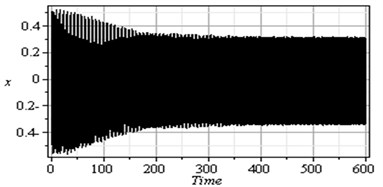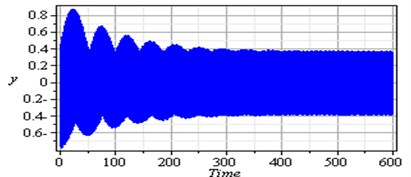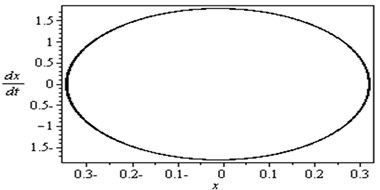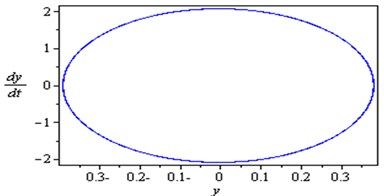To determine the numerical solution and response of the given system of Eq. (4), the Runge-Kutta of fourth order method was applied. Fig. 2 illustrates the response and the phase-plane for the non-resonant system (basic case) where $\text{\hspace{0.17em}}{\gamma }_{1}\ne {\gamma }_{2}\ne {\gamma }_{3}\ne {\gamma }_{4}\ne {\omega }_{1}\ne {\omega }_{2}$ at some practical values of the equation parameters ${\mu }_{1}=\text{0.02}$, ${\beta }_{1}=\text{0.03}$, ${\beta }_{2}=\text{0.3}$, ${\beta }_{3}=\text{0.2}$, ${\beta }_{4}=\text{0.05}$, ${\beta }_{5}=\text{0.3}$, ${\mu }_{2}=\text{0.02}$, ${\eta }_{1}=\text{0.3}$, ${\eta }_{2}=\text{0.03}$, ${\eta }_{3}=\text{0.5}$, ${\eta }_{4}=\text{0.03}$, ${\eta }_{5}=\text{0.3}$, ${\rho }_{1}=\text{8.6}$, ${\rho }_{2}=\text{8.6}$, ${\rho }_{11}=\text{0.02}$, ${\rho }_{12}=\text{0.02}$, ${\rho }_{21}=\text{0.005}$, ${\rho }_{22}=\text{0.005}$, ${\eta }_{1}=\text{0.3}$, $\text{\hspace{0.17em}}{\eta }_{2}=\text{0.03}$, ${\eta }_{3}=\text{0.5}$, ${\eta }_{4}=\text{0.03}$, ${\eta }_{5}=\text{0.3}$, ${\rho }_{1}=\text{8.6}$, ${\rho }_{2}=\text{8.6}$, ${\rho }_{11}=\text{0.02}$, ${\rho }_{12}=\text{0.02}$, ${\rho }_{21}=\text{0.005}$, ${\rho }_{22}=\text{0.005}$. It is observed from this figure that the response of the first and second modes of the FGM rectangular plate start with increasing amplitude with some chaotic and tuned oscillation respectively, the oscillation of the two modes becomes stable and the steady state amplitudes $x$ and $y$ are about 0.35 and 0.4 respectively and the phase plane shows limit cycle, denoting that the system is free from chaos. Table 1 shows the results of the worst resonance conditions.

Fig. 3 shows that the time response and phase-plane of the simultaneous primary, combined and internal resonance (${\gamma }_{1}\cong {\omega }_{1}$, $\text{\hspace{0.17em}}{\gamma }_{3}-{\gamma }_{4}\cong {\omega }_{1}$ and ${\omega }_{2}\cong 1/2{\omega }_{1}$) which is one of the worst resonance cases. From this figure we have that the amplitude of the first mode of the FGM rectangular plate is increased to about 1950 % of that values shown in Fig. 2, while the amplitude of the second mode is increased to about 1150 % and becomes stable and the phase plane shows multi-limit cycle.

Fig. 3Simultaneous primary combined and internal resonance case: μ1=0.02, β1=0.03, β2=0.3, β3=0.2, β4=0.05, β5=0.3, μ2=0.02, η1=0.3, η2=0.03, η3=0.5, η4=0.03, η5=0.3, ρ1=8.6, ρ2=8.6, ρ11=0.02, ρ12=0.02, ρ21=0.005, ρ22=0.005 ( γ1≅ω1,  γ3-γ4≅ω1 and ω2≅1/2ω1)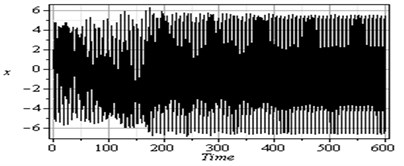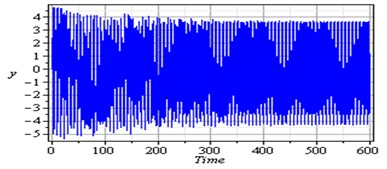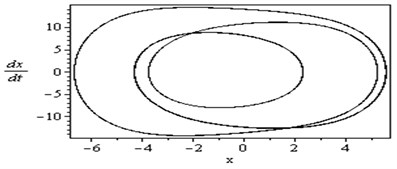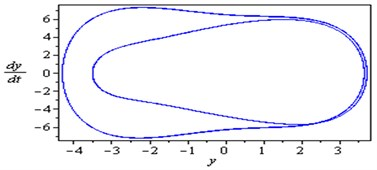Table 1Summary of the worst resonance cases

 Resonance cases $x$ % $y$ % Remarks Non-resonance case 100 % 100 % Limit cycle ${\gamma }_{1}\cong {\omega }_{1}\text{,}\text{}{\gamma }_{1}\cong {\omega }_{2}\text{,}\text{}{\omega }_{2}\cong {\omega }_{1}$ 1450 % 1000 % Multi Limit cycle ${\gamma }_{1}\cong {\omega }_{1}\text{,}\text{}{\gamma }_{2}\cong 2{\omega }_{2}\text{,}\text{}{\omega }_{2}\cong {\omega }_{1}$ 1450 % 900 % Multi Limit cycle ${\gamma }_{1}\cong {\omega }_{1}\text{,\hspace{0.17em}}{\gamma }_{3}-{\gamma }_{4}\cong {\omega }_{2}\text{,\hspace{0.17em}}{\omega }_{2}\cong {\omega }_{1}$ 1450 % 1000 % Multi Limit cycle ${\gamma }_{1}\cong {\omega }_{2}\text{,\hspace{0.17em}}{\gamma }_{2}\cong 2{\omega }_{1}\text{,\hspace{0.17em}}{\omega }_{2}\cong {\omega }_{1}$ 1450 % 900 % Multi Limit cycle ${\gamma }_{1}\cong {\omega }_{2}\text{,\hspace{0.17em}}{\gamma }_{3}-{\gamma }_{4}\cong {\omega }_{1}\text{,\hspace{0.17em}}{\omega }_{2}\cong {\omega }_{1}$ 1450 % 1000 % Multi Limit cycle ${\gamma }_{1}\cong {\omega }_{1}\text{,\hspace{0.17em}}{\gamma }_{1}\cong {\omega }_{2}\text{,}\text{}{\gamma }_{3}-{\gamma }_{4}\cong {\omega }_{1}$ 1450 % 1000 % Multi Limit cycle ${\gamma }_{1}\cong {\omega }_{1}\text{,\hspace{0.17em}}{\gamma }_{2}\cong 2{\omega }_{2}\text{,\hspace{0.17em}}{\gamma }_{3}-{\gamma }_{4}\cong {\omega }_{1}$ 1400 % 900 % Multi Limit cycle ${\gamma }_{1}\cong {\omega }_{2}\text{,\hspace{0.17em}}{\gamma }_{2}\cong 2{\omega }_{1}\text{,\hspace{0.17em}}{\gamma }_{3}-{\gamma }_{4}\cong {\omega }_{1}$ 1450 % 800 % Multi Limit cycle ${\gamma }_{1}\cong {\omega }_{1}\text{,\hspace{0.17em}}{\gamma }_{3}-{\gamma }_{4}\cong {\omega }_{1}\text{,}\text{}{\omega }_{2}\cong 1/2{\omega }_{1}$ 1950 % 1150 % Multi Limit cycle ${\gamma }_{1}\cong {\omega }_{1}\text{,\hspace{0.17em}}{\gamma }_{3}-{\gamma }_{4}\cong {\omega }_{1}\text{,}\text{}{\omega }_{2}\cong 1/3{\omega }_{1}$ 1900 % 1100 % Multi Limit cycle ${\gamma }_{1}\cong {\omega }_{1}\text{,\hspace{0.17em}}{\gamma }_{3}-{\gamma }_{4}\cong {\omega }_{1}\text{,}\text{}{\omega }_{2}\cong 1/4{\omega }_{1}$ 1900 % 1100 % Multi Limit cycle

### 4.1. Response curves and effects of different parameters

In this section, the steady state response of the given system at various parameters near the simultaneous primary, combined and internal resonance case is investigated and studied. The frequency response equations given by Eq. (26) are solved numerically at the same values of the parameters shown in Fig. 3. In all figures, the solid lines stand for the stable solution and the dashed lines for the unstable solution.

Fig. 4The steady state amplitudes of the first mode of the FGM rectangular plate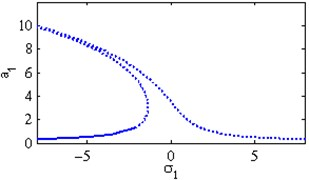a) Effects of the detuning parameter ${\sigma }_{1}$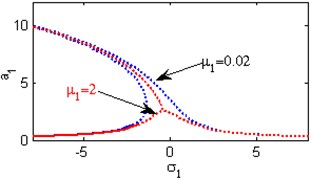b) Effects of the damping coefficient ${\mu }_{1}$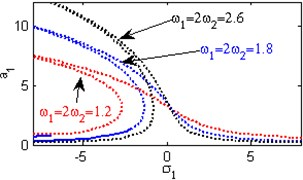c) Effect of the natural frequencies ${\omega }_{1}$, ${\omega }_{2}$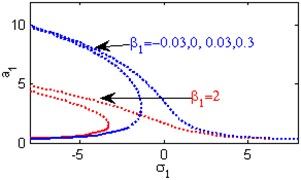d) Effects of the non-linear parameter ${\beta }_{1}$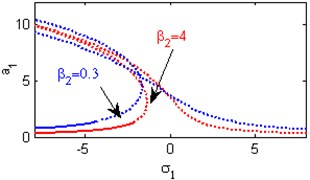e) Effects of the non-linear parameter ${\beta }_{2}$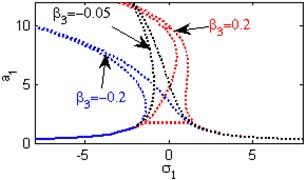f) Effects of the non-linear parameter ${\beta }_{3}$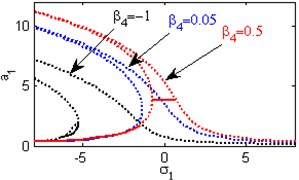g) Effects of the non-linear parameter ${\beta }_{4}$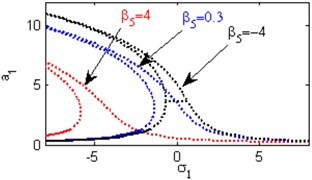h) Effects of the non-linear parameter ${\beta }_{5}$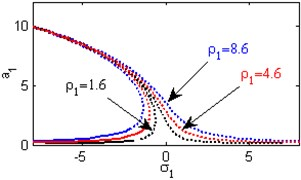i) Effects of the excitation amplitude ${\rho }_{1}$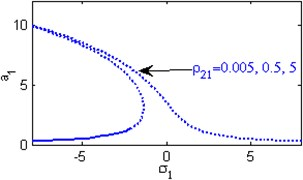j) Effects of the excitation amplitude ${\rho }_{21}$

Fig. 4(a) shows the steady state amplitudes of the first mode of the FGM rectangular plate against the detuning parameter ${\sigma }_{1}$ at the practical case, where ${a}_{1}\ne \text{0}$, ${a}_{2}\text{\hspace{0.17em}}\ne \text{0}$. Fig. 4(b) shows that the steady state amplitude of the first mode of the FGM rectangular plate is a monotonic decreasing function in the linear damping coefficients ${\mu }_{1}$. Fig. 4(c) show that the steady state amplitude of the first mode is a monotonic decreasing function in the natural frequencies ${\omega }_{1}$ and ${\omega }_{2}$, in this Figure, the response curve is bent to the right and has harding spring type and there exists jump phenomena. Figs. 4(d), (e) shows that the steady state amplitude of the first mode is a monotonic decreasing function in nonlinear parameter ${\beta }_{1}$,${\beta }_{2}$ and the response curves are bent to the left leading to the occurrence of the jump phenomena and multi-valued amplitudes. The steady state amplitude of the first mode is a monotonic increasing function in the nonlinear parameter ${\beta }_{3}$, ${\beta }_{4}$ and the excitation amplitudes ${\rho }_{1}$ as shown in Figs. 4(f), (g), (i). For increasing value of the nonlinear parameter ${\beta }_{5}$, the amplitude of the first mode is decreased and the curve is shifted to the left as shown in Fig. 4(h). The effect of increasing or decreasing the excitation amplitudes ${\rho }_{21}$ on the steady state amplitude of the FGM is trivial due to saturation occurrence as shown in Fig. 4(j).

Fig. 5The steady state amplitudes of the second mode of the FGM rectangular plate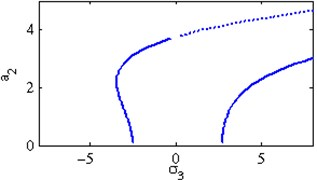a) Effects of the detuning parameter ${\sigma }_{3}$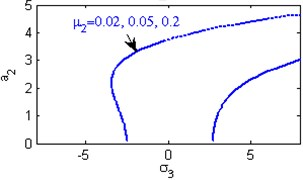b) Effects of the damping coefficient ${\mu }_{2}$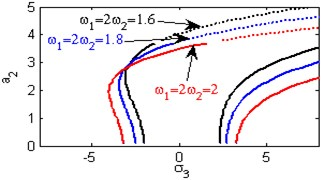c) Effect of the natural frequencies ${\omega }_{1}$, ${\omega }_{2}$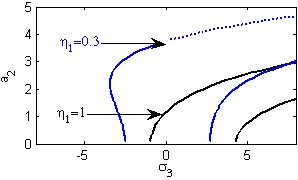d) Effects of the non-linear parameter ${\eta }_{1}$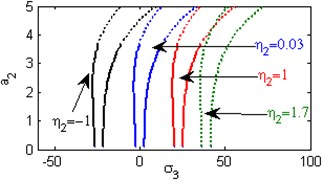e) Effects of the non-linear parameter ${\eta }_{2}$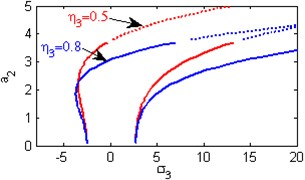f) Effects of the non-linear parameter ${\eta }_{3}$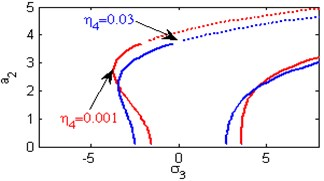g) Effects of the non-linear parameter ${\eta }_{4}$h) Effects of the non-linear parameter ${\eta }_{5}$

Fig. 5(a) shows the steady state amplitudes of the second mode of the FGM rectangular plate against the detuning parameter ${\sigma }_{3}$ at the practical case, where ${a}_{1}\ne \text{0}$, ${a}_{2}\ne \text{0}$. In this Figure, the response amplitude consists of a continuous curve which is bent to the right and there exists jump phenomena. Fig. 5(b) shows that the effect of increasing or decreasing the linear damping coefficients ${\mu }_{2}$ on the steady state amplitude of the second mode of FGM is trivial due to saturation occurrence. Figs. 5(c), (d), (f), (g) show that the steady state amplitude of the second mode is a monotonic decreasing function in nonlinear parameter ${\eta }_{1}$,${\eta }_{3}$, ${\eta }_{4}$ and the natural frequencies ${\omega }_{1}$, ${\omega }_{2}$ and the response curves are bent to the right leading to the occurrence of the jump phenomena and multi-valued amplitudes. For increasing value of the nonlinear parameter ${\eta }_{2}$, the amplitude of the second mode is shifted to the right as shown in Fig. 5(e). The steady state amplitude of the first mode is a monotonic increasing function in the nonlinear parameter ${\eta }_{5}$, as shown in Fig. 5(h).

### 4.2. Comparison between the numerical and analytical simulation

Fig. 6Comparison between numerical solution (using RKM) and analytical solution (using perturbation method) of the system at ρ1=8.6, ρ2=8.6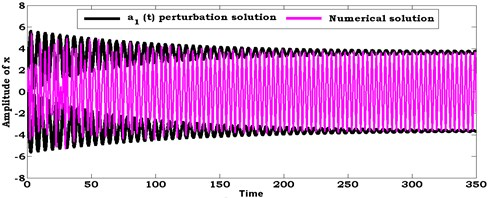a)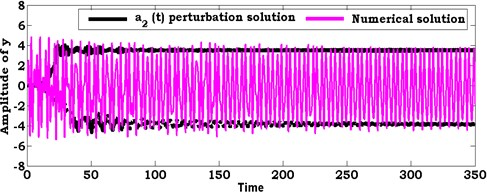b)

Fig. 7Comparison between numerical solution (using RKM) and analytical solution (using perturbation method) of the system at ρ1=1.6, ρ2=1.6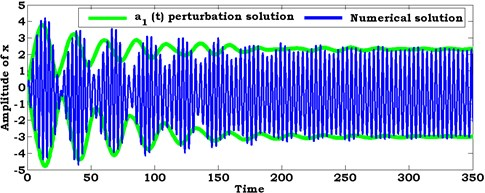a)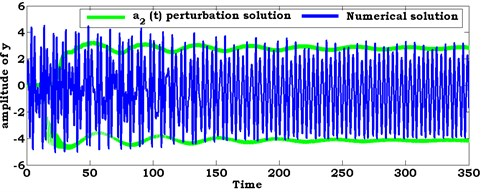b)

In this section, the system of nonlinear differential equations given by Eqs. (21) and (22) are solved numerically at the simultaneous primary, combined and internal resonance case where ${\gamma }_{1}\cong {\omega }_{1}$, $\text{\hspace{0.17em}}{\gamma }_{3}-{\gamma }_{4}\cong {\omega }_{1}$ and ${\omega }_{2}\cong 1/2{\omega }_{1}$. Figs. 6 and 7 show the comparison between numerical integration for the system Eqs. 4(a), (b) and the amplitude-phase modulating Eqs. (21) and (22) at different values of excitation force amplitudes ${\rho }_{1}$ and ${\rho }_{2}$. We found that all predictions from analytical solutions are in good agreement with the numerical simulation.

## 5. Conclusions

Multiple time scale perturbation method is useful to determine approximate solutions for the coupled nonlinear differential equations describing the FGM rectangular plate system up to and including the second order approximation. Both the frequency response equations and the phase-plane technique are applied to study the stability of the system. The effect of the different parameters of the system is studied numerically. From the above study the following may be concluded:

1) The amplitude of the first mode FGM rectangular plate is increased to about 0.35, while the amplitude of the second mode is increased to about 0.4 at the non-resonant case (${\gamma }_{1}\ne {\gamma }_{2}\ne {\gamma }_{3}\ne {\gamma }_{4}\ne {\omega }_{1}\ne {\omega }_{2}$).

2) The amplitude of the first mode FGM rectangular plate is increased to about 1950 % of that values shown in Fig. 2, while the amplitude of the second mode is increased to about 1150 % at the worst resonance case (the simultaneous primary, combined and internal resonance $\text{\hspace{0.17em}}{\gamma }_{1}\cong {\omega }_{1}$, ${\gamma }_{3}-{\gamma }_{4}\cong {\omega }_{1}$ and ${\omega }_{2}\cong 1/2{\omega }_{1}$).

3) The amplitude of the first and second mode FGM rectangular plate are increased to about 1900 % and 1100 % respectively at the worst resonance case (${\gamma }_{1}\cong {\omega }_{1}$, ${\gamma }_{3}-{\gamma }_{4}\cong {\omega }_{1}$ and ${\omega }_{2}\cong 1/3{\omega }_{1}$) and (${\gamma }_{1}\cong {\omega }_{1}$, ${\gamma }_{3}-{\gamma }_{4}\cong {\omega }_{1}$ and ${\omega }_{2}\cong 1/4{\omega }_{1}$).

4) The steady state amplitudes of the FGM rectangular plate are a monotonic decreasing function the linear damping coefficients ${\mu }_{1}$, the nonlinear parameters (${\beta }_{1}$, ${\beta }_{2}$, ${\beta }_{5}$, ${\eta }_{1}$, ${\eta }_{3}$, ${\eta }_{4}$) and the natural frequencies ${\omega }_{1}$ and ${\omega }_{2}$.

5) The steady state amplitudes of FGM rectangular plate are a monotonic increasing function in the nonlinear parameters (${\beta }_{3}$, ${\beta }_{4}$, ${\eta }_{5}$) and the excitation amplitude $\text{\hspace{0.17em}}{\rho }_{1}$.

6) The effects of increasing or decreasing the linear damping coefficients ${\mu }_{2}$ and the excitation amplitude ${\rho }_{21}$ on the steady state amplitude of the FGM are trivial due to saturation occurrence.

7) The analytical solutions are in good agreement with the numerical simulation at different values of excitation amplitude forces ${\rho }_{1}$ and ${\rho }_{2}$.

03 December 2013
Accepted
20 June 2014
Published
15 November 2014
Keywords
FGM rectangular plate
vibrations
chaotic dynamics
stability
saturation
Acknowledgements

The author would like to thank the anonymous reviewers for their valuable comments and suggestions for improving the quality of this paper.